# Chapter 5 Spatial Data and MapsThe Spatial section of this book adds the spatial dimension and geographic data science. Most environmental systems are significantly affected by location, so the geographic perspective is highly informative. In this chapter, we’ll explore the basics of

• building and using feature-based (vector) data using the `sf` (simple features) package
• raster data using the `terra` package
• some common mapping methods in `plot` and `ggplot2`

Especially for the feature data which is built on a database model (with geometry as one variable), the tools we’ve been learning in `dplyr` and `tidyr` will also be useful to working with attributes.

Caveat: Spatial data and analysis is very useful for environmental data analysis, and we’ll only scratch the surface. Readers are encouraged to explore other resources on this important topic, such as Geocomputation with R at https://geocompr.robinlovelace.net/ or https://r-spatial.github.io/sf/ .

## 5.1 Spatial Data (and Geospatial Data)

Spatial data are data using a Cartesian coordinate system with x, y, z, and maybe more dimensions. Geospatial data are spatial data that we can map on our planet and relate to other geospatial data based on geographic coordinate systems (GCS) of longitude and latitude or known projections to planar coordinates like Mercator, Albers, or many others. You can also use local coordinate systems with the methods we’ll learn, to describe geometric objects or a local coordinate system to “map out” an archaeological dig or describe the movement of ants on an anthill, but we’ll primarily use geospatial data.

To work with spatial data requires extending R to deal with it using packages. Many have been developed, but the field is starting to mature using international open GIS standards.

`sp` (until recently, the dominant library of spatial tools)

• Includes functions for working with spatial data
• Includes `spplot` to create maps
• Also needs `rgdal` package for `readOGR` – reads spatial data frames
• Earliest archived version on CRAN is 0.7-3, 2005-04-28, with 1.0 released 2012-09-30. Authors: Edzer Pebesma & Roger Bivand

`sf` (Simple Features)

• Feature-based (vector) methods
• ISO 19125 standard for GIS geometries
• Also has functions for working with spatial data, but clearer to use.
• Doesn’t need many additional packages, though you may still need `rgdal` installed for some tools you want to use.
• Replacing `sp` and `spplot` though you’ll still find them in code. We’ll give it a try…
• Works with ggplot2 and tmap for nice looking maps.
• Cheat sheet: https://github.com/rstudio/cheatsheets/raw/master/sf.pdf
• Earliest CRAN archive is 0.2 on 2016-10-26, 1.0 not until 2021-06-29.
• Author: Edzer Pebesma

`raster`

• The established raster package for R, with version 1.0 from 2010-03-20, Author: Robert J. Hijmans (Professor of Environmental Science and Policy at UC Davis)
• Depends on `sp`
• CRAN: “Reading, writing, manipulating, analyzing and modeling of spatial data. The package implements basic and high-level functions for raster data and for vector data operations such as intersections. See the manual and tutorials on https://rspatial.org/ to get started.”

`terra`

• Intended to eventually replace `raster`, by the same author (Hijmans), with version 0.5-2 2020-03-20, and 1.0 2021-01-04. Does not depend on `sp`, but includes Bivand and Pebesma as contributors.
• CRAN: “Methods for spatial data analysis with raster and vector data. Raster methods allow for low-level data manipulation as well as high-level global, local, zonal, and focal computation. The predict and interpolate methods facilitate the use of regression type (interpolation, machine learning) models for spatial prediction, including with satellite remote sensing data. Processing of very large files is supported. See the manual and tutorials on https://rspatial.org/terra/ to get started.”

### 5.1.1 Simple geometry building in sf

Simple Features (`sf`) includes a set of simple geometry building tools that use matrices or lists of matrices of XYZ (or just XY) coordinates (or lists of lists for multipolygon) as input, and each basic geometry type having single and multiple versions (Table 5.1).

TABLE 5.1: Some common sf functions for building geometries from coordinates
sf function input
st_point() numeric vector of length 2, 3, or 4, represent a single point
st_multipoint() numeric matrix with points in rows
st_linestring() numeric matrix with points in rows
st_multilinestring() list of numeric matrices with points in rows
st_polygon() list of numeric matrices with points in rows
st_multipolygon() list of lists with numeric matrices

sf functions have the pattern `st_*` (st means “space and time”)

See Geocomputation with R at https://geocompr.robinlovelace.net/ or https://r-spatial.github.io/sf/ for more details, but here’s an example of manual feature creation of sf geometries (sfg) followed by building a simple feature geometry list column from the collection with `st_sfc()`:

``````library(tidyverse)
library(sf)
library(igisci)``````

#### 5.1.1.1 Building simple sf geometries

We’ll build some simple geometries and plot them, just to illustrate the types of geometries. Try the following code.

``````library(sf); library(tidyverse)
eyes <- st_multipoint(rbind(c(1,5), c(3,5)))
nose <- st_point(c(2,4))
mouth <- st_linestring(rbind(c(1,3),c(3, 3)))
border <- st_polygon(list(rbind(c(0,5), c(1,2), c(2,1), c(3,2),
c(4,5), c(3,7), c(1,7), c(0,5))))
plot(st_sfc(eyes, nose, mouth, border))``````

#### 5.1.1.2 Building geospatial points as sf columns (`sfc`)

The face-building example above is really intended for helping to understand the concept and isn’t really how we’d typically build data. For one thing, it doesn’t have a real coordinate system that can be reprojected into locations anywhere on the planet. And most commonly we’ll be accessing data that have been created by government agencies such as those from the USGS in The National Map, or NGO, university or corporate sources who have an interest in collecting and providing data.

However, let’s take the `sfc` building a bit further to better understand it, and build mappable sf columns (sfc) from scratch.

We’ll build a generalized map of California and Nevada boundaries (and then later in the exercises, you’ll expand this to a map of the west.) We’ll use a shortcut to the coordinate referencing system (crs) by using a short numeric EPSG code (EPSG Geodetic Parameter Dataset (n.d.)), 4326, for the common geographic coordinate system (GCS) of latitude and longitude.

``````library(sf)
CA_matrix <- rbind(c(-124,42),c(-120,42),c(-120,39),c(-114.5,35),
c(-114.1,34.3),c(-114.6,32.7),c(-117,32.5),c(-118.5,34),c(-120.5,34.5),
c(-122,36.5),c(-121.8,36.8),c(-122,37),c(-122.4,37.3),c(-122.5,37.8),
c(-123,38),c(-123.7,39),c(-124,40),c(-124.4,40.5),c(-124,41),c(-124,42))
NV_matrix <- rbind(c(-120,42),c(-114,42),c(-114,36),c(-114.5,36),
c(-114.5,35),c(-120,39),c(-120,42))
CA_list <- list(CA_matrix);       NV_list <- list(NV_matrix)
CA_poly <- st_polygon(CA_list);   NV_poly <- st_polygon(NV_list)
sfc_2states <- st_sfc(CA_poly,NV_poly,crs=4326)  # crs=4326 specifies GCS
st_geometry_type(sfc_2states)``````
``````##  POLYGON POLYGON
## 18 Levels: GEOMETRY POINT LINESTRING POLYGON MULTIPOINT ... TRIANGLE``````

Then we’ll use the `geom_sf()` function in ggplot2 to create a map (Figure 5.1).

``````library(tidyverse)
ggplot() + geom_sf(data = sfc_2states)``````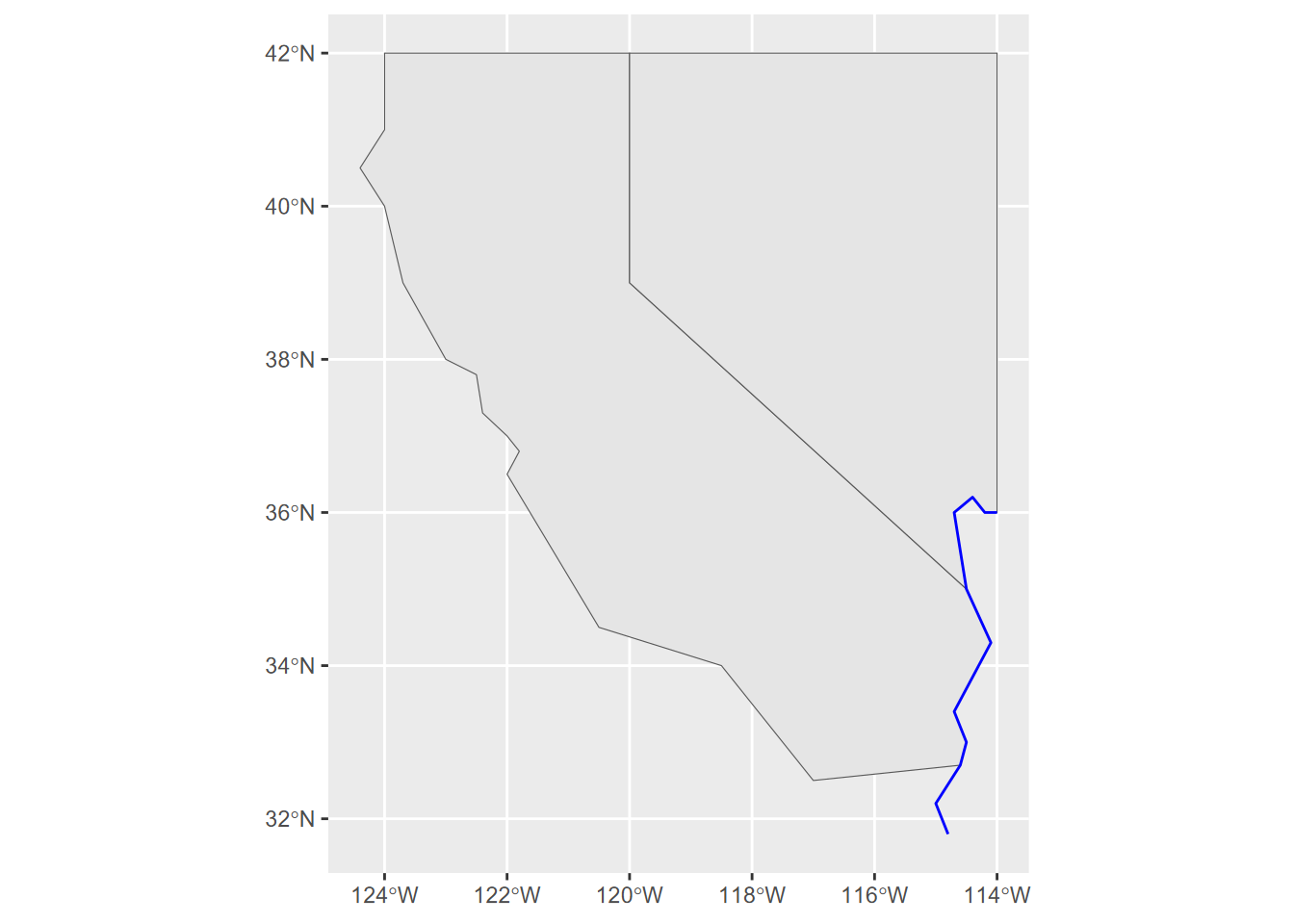FIGURE 5.1: A simple ggplot2 map built from scratch with hard-coded data as simple feature columns

#### 5.1.1.3 Building an sf class from sf columns.

So far, we’ve built an `sfg` (sf geometry) and then a collection of matrices in an `sfc` (sf column), and we were even able to make a map of it. But to be truly useful, we’ll want other attributes other than just where the feature is, so we’ll build an `sf` that has attributes, and that can also be used like a data frame – similar to a shapefile, since a data frame is like a database table `.dbf`. We’ll build one with the `st_sf()` function from attributes and geometry (Figure 5.2).

``````attributes <- bind_rows(c(abb="CA", area=423970, pop=39.56e6),
c(abb="NV", area=286382, pop=3.03e6))
twostates <- st_sf(attributes, geometry = sfc_2states)
ggplot(twostates) + geom_sf() + geom_sf_text(aes(label = abb))``````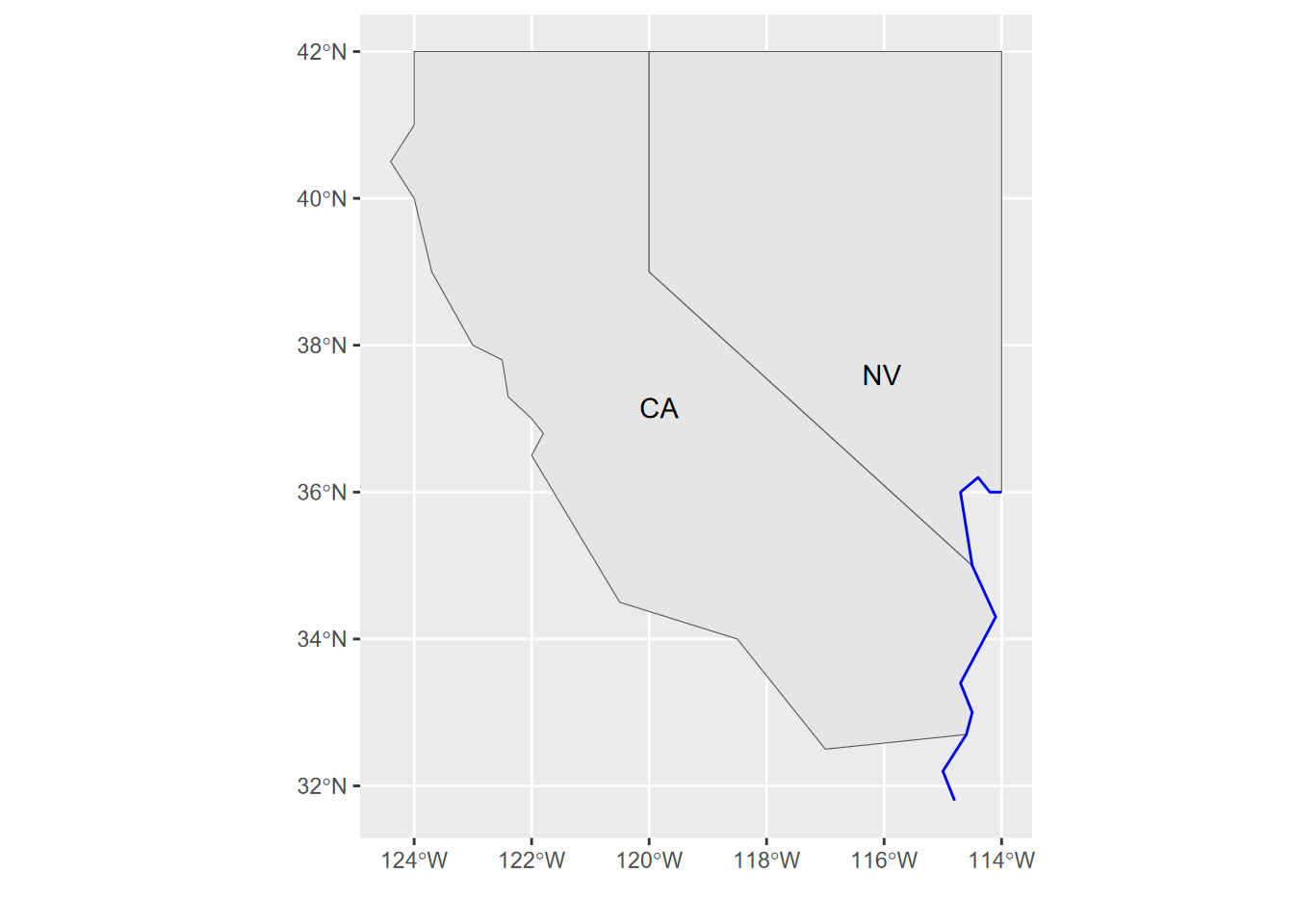FIGURE 5.2: Using an sf class to build a map in ggplot2, displaying an attribute

Or we could let the plot system try to figure out what to do with it. To help it out a bit, we can select just one variable, for which we need to use the `[]` accessor (Figure 5.3). Try the `\$` accessor instead to see what you get, and consider why this might be.

``plot(twostates["abb"])``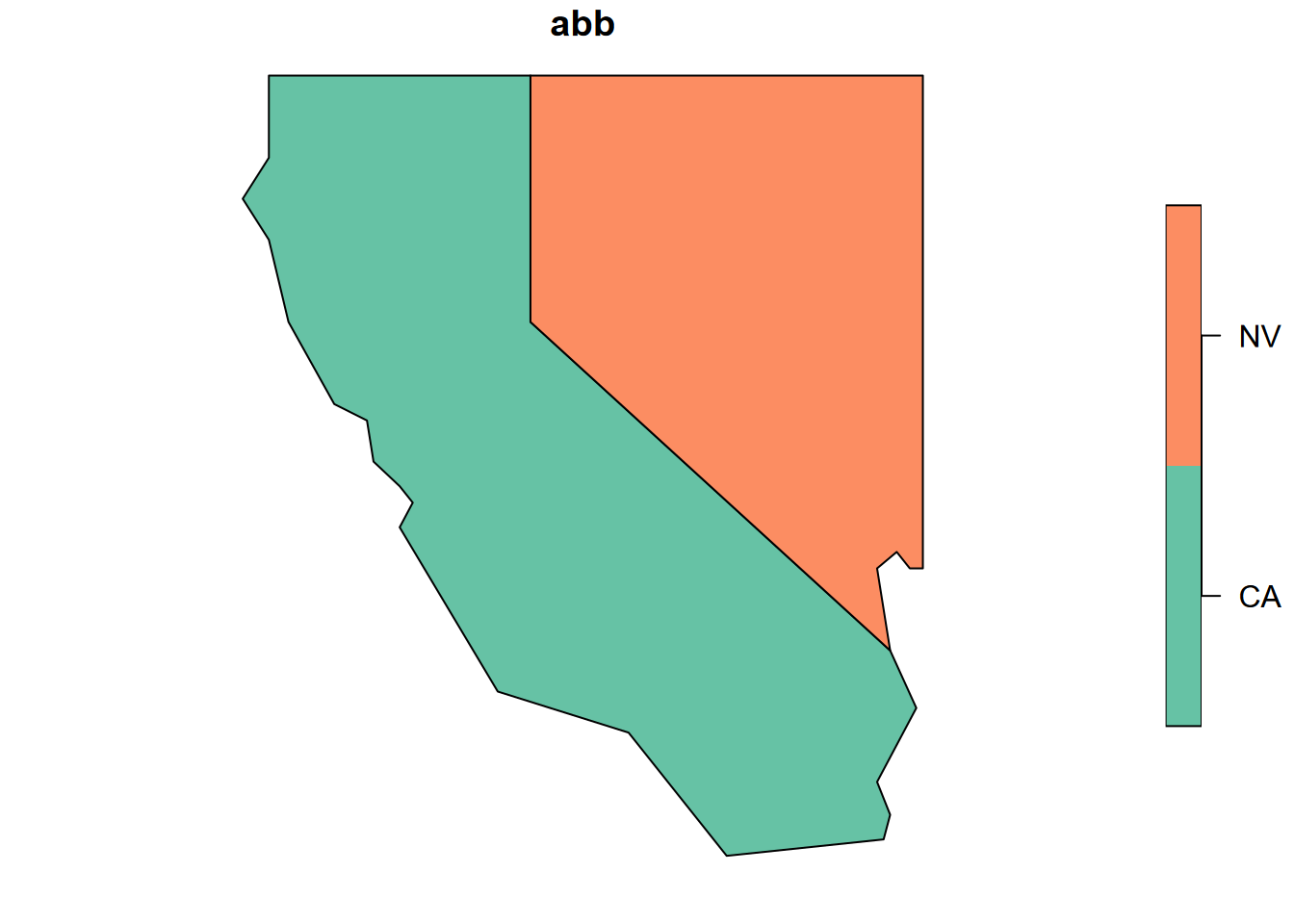FIGURE 5.3: Base R plot of one attribute from two states

### 5.1.2 Building points from a data frame

We’re going to take a different approach with points, since if we’re building them from scratch they commonly come in the form of a data frame with x and y columns, so the `st_as_sf` is much simpler than what we just did with `sfg` and `sfc` data.

The `sf::st_as_sf` function is useful for a lot of things, and follows the convention of R’s many “as” functions: if it can understand the input as having “space and time” (st) data, it can convert it to an sf.

We’ll start by creating a data frame representing 12 Sierra Nevada (and some adjacent) weather stations, with variables entered as vectors, including attributes. The order of vectors needs to be the same for all, where the first point has index 1, etc.

``````sta  <- c("OROVILLE","AUBURN","PLACERVILLE","COLFAX","NEVADA CITY","QUINCY",
"YOSEMITE","PORTOLA","TRUCKEE","BRIDGEPORT","LEE VINING","BODIE")
long <- c(-121.55,-121.08,-120.82,-120.95,-121.00,-120.95,
-119.59,-120.47,-120.17,-119.23,-119.12,-119.01)
lat <-  c(39.52,38.91,38.70,39.09,39.25,39.94,
37.75,39.81,39.33,38.26,37.96,38.21)
elev <- c(52,394,564,725,848,1042,
1225,1478,1775,1972,2072,2551)
temp <- c(10.7,9.7,9.2,7.3,6.7,4.0,
5.0,0.5,-1.1,-2.2,0.4,-4.4)
prec <- c(124,160,171,207,268,182,
169,98,126,41,72,40)``````

To build the sf data, we start by building the data frame…

``df <- data.frame(sta,elev,temp,prec,long,lat)``

… then use the very handy `sf::st_as_sf` function to make an sf out of it, using the long and lat as a source of coordinates (Figure 5.4).

``````wst <- st_as_sf(df, coords=c("long","lat"), crs=4326)
ggplot(data=wst) + geom_sf(mapping = aes(col=elev))``````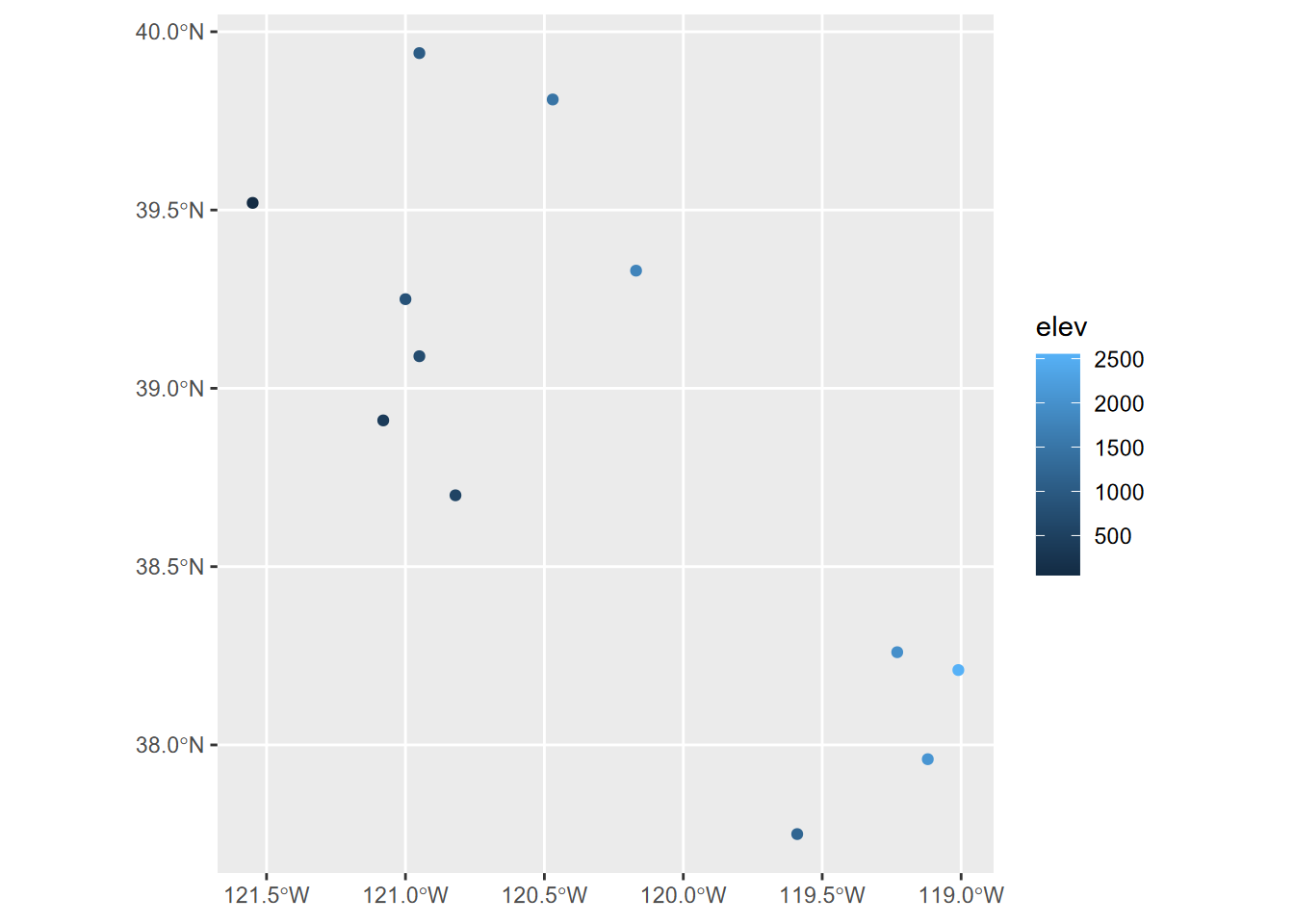FIGURE 5.4: Points created from a dataframe with Simple Features

### 5.1.3 SpatVectors in `terra`

We’ll briefly look at another feature-based object format, the `SpatVector` from `terra`, which uses a formal S4 object definition which will provide some advantages over S3 objects such as what’s in most of R, at the expense of being a bit less flexible and not compatible with some packages, at least at the time of writing; that will probably change. See Hijmans (n.d.) for more about SpatVectors. We’ll use terra’s raster capabilities a lot more, but we’ll take a brief look at building and using a `SpatVector`.

Then we’ll build a `SpatVector` we’ll call `station_pts` from just the longitude and latitude vectors we just created:

``````library(terra)
longlat <- cbind(long,lat)
station_pts <- vect(longlat)``````

Unlike other data sets which use a simple object model called S3, `SpatVector` data use the more formal S4 definition. SpatVectors have a rich array of properties and associated methods, which you can see in the Environment tab of RStudio, or access with `@ptr`. This is a good thing, but the `str()` function we’ve been using for S3 objects presents us with arguably too much information for easy understanding. But just by entering `station_pts` on the command line we get something similar to what `str()` does with S3 data, so this might be better for seeing a few key properties:

``station_pts``
``````##  class       : SpatVector
##  geometry    : points
##  dimensions  : 12, 0  (geometries, attributes)
##  extent      : -121.55, -119.01, 37.75, 39.94  (xmin, xmax, ymin, ymax)
##  coord. ref. :``````

Note that there’s nothing listed for `coord. ref.` meaning the coordinate referencing system (CRS). We’ll look at this more below, but for now let’s change the code a bit to add this information using the PROJ-string method:

``station_pts <- vect(longlat, crs = "+proj=longlat +datum=WGS84")``

Of the many methods associated with these objects, one example is getting with geom():

``geom(station_pts)``
``````##       geom part       x     y hole
##  [1,]    1    1 -121.55 39.52    0
##  [2,]    2    1 -121.08 38.91    0
##  [3,]    3    1 -120.82 38.70    0
##  [4,]    4    1 -120.95 39.09    0
##  [5,]    5    1 -121.00 39.25    0
##  [6,]    6    1 -120.95 39.94    0
##  [7,]    7    1 -119.59 37.75    0
##  [8,]    8    1 -120.47 39.81    0
##  [9,]    9    1 -120.17 39.33    0
## [10,]   10    1 -119.23 38.26    0
## [11,]   11    1 -119.12 37.96    0
## [12,]   12    1 -119.01 38.21    0``````

Let’s add the attributes to the original `longlat` matrix to build a SpatVect with attributes we can see in a basic plot (Figure 5.5).

``````df <- data.frame(sta,elev,temp,prec)
clim <- vect(longlat, atts=df, crs="+proj=longlat +datum=WGS84")
clim``````
``````##  class       : SpatVector
##  geometry    : points
##  dimensions  : 12, 4  (geometries, attributes)
##  extent      : -121.55, -119.01, 37.75, 39.94  (xmin, xmax, ymin, ymax)
##  coord. ref. : +proj=longlat +datum=WGS84 +no_defs
##  names       :         sta  elev  temp  prec
##  type        :       <chr> <num> <num> <num>
##  values      :    OROVILLE    52  10.7   124
##                     AUBURN   394   9.7   160
##                PLACERVILLE   564   9.2   171``````
``````plot(clim)
text(clim, labels="sta", pos=4)``````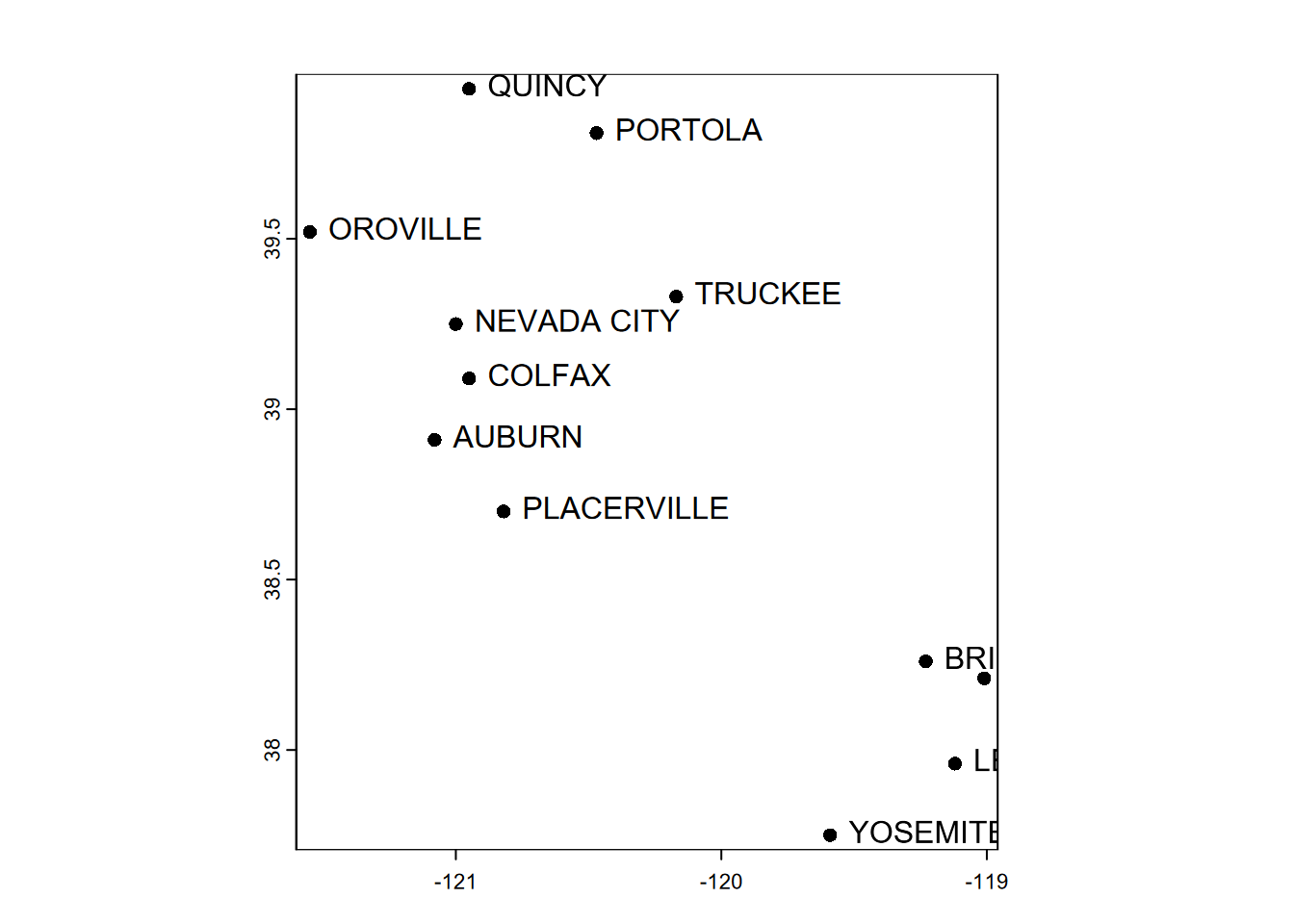FIGURE 5.5: Simple plot of SpatVector point data, with labels

The SpatVector format looks promising, but sometimes you may want an sf instead. Fortunately, you can use the `sf::sf_as_sf` function to convert it, just as you can create a `SpatVector` from an `sf` with the `terra::vect` function. The following code demonstrates both, with a ggplot2 map of sf data (Figure 5.6) and then a base R plot map with SpatVector data (Figure 5.7).

``````library(sf); library(ggplot2)
climsf <- st_as_sf(clim)
str(climsf)``````
``````## Classes 'sf' and 'data.frame':   12 obs. of  5 variables:
##  \$ sta     : chr  "OROVILLE" "AUBURN" "PLACERVILLE" "COLFAX" ...
##  \$ elev    : num  52 394 564 725 848 ...
##  \$ temp    : num  10.7 9.7 9.2 7.3 6.7 4 5 0.5 -1.1 -2.2 ...
##  \$ prec    : num  124 160 171 207 268 182 169 98 126 41 ...
##  \$ geometry:sfc_POINT of length 12; first list element:  'XY' num  -121.5 39.5
##  - attr(*, "sf_column")= chr "geometry"
##  - attr(*, "agr")= Factor w/ 3 levels "constant","aggregate",..: NA NA NA NA
##   ..- attr(*, "names")= chr [1:4] "sta" "elev" "temp" "prec"``````
``ggplot() + geom_sf(data=twostates) + geom_sf(data=climsf)``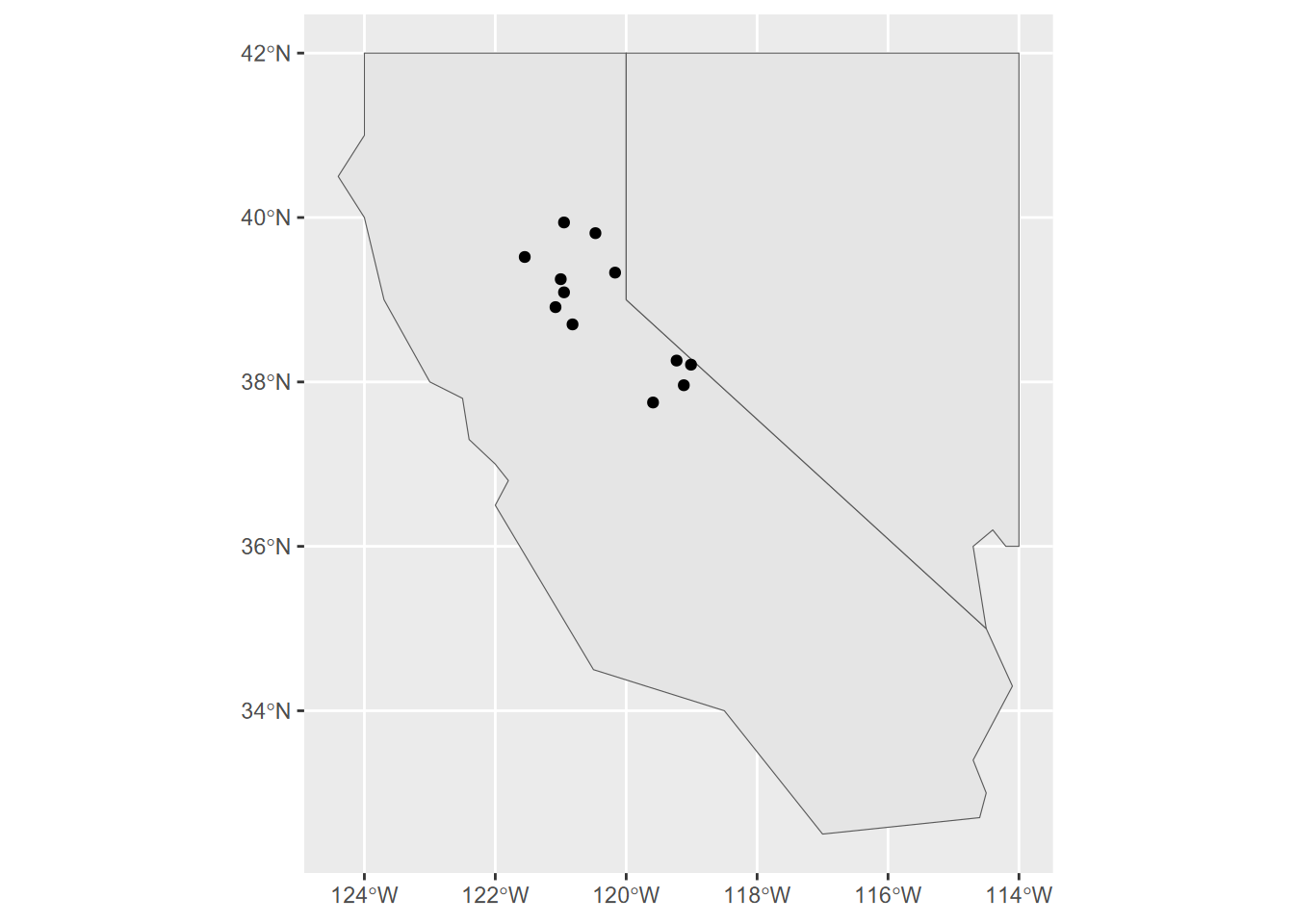FIGURE 5.6: ggplot of twostates and stations

``````twostatesV <- vect(twostates)
plot(twostatesV)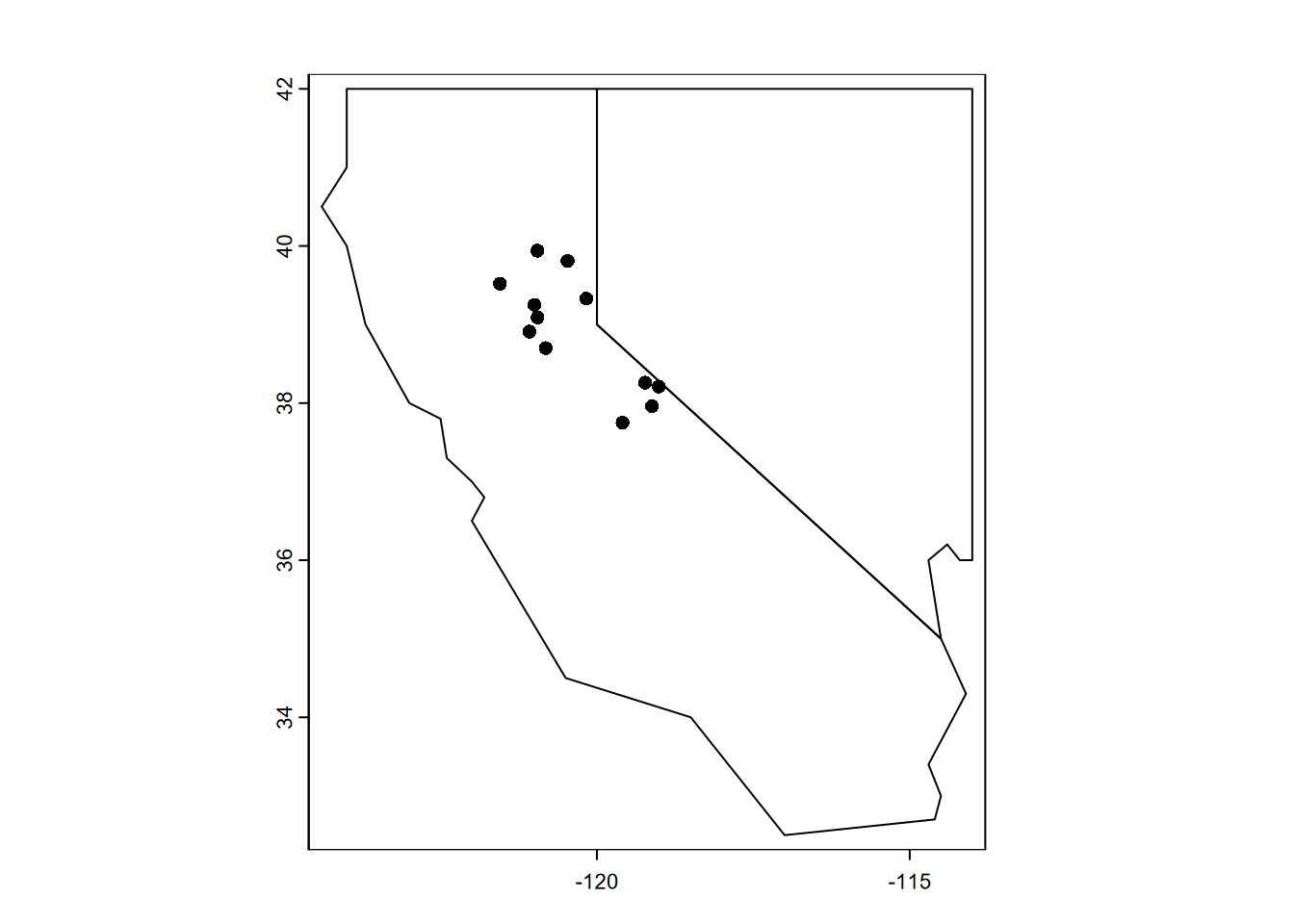FIGURE 5.7: Base R plot of twostates and stations SpatVectors

``twostatesV``
``````##  class       : SpatVector
##  geometry    : polygons
##  dimensions  : 2, 3  (geometries, attributes)
##  extent      : -124.4, -114, 32.5, 42  (xmin, xmax, ymin, ymax)
##  coord. ref. : lon/lat WGS 84 (EPSG:4326)
##  names       :   abb   area      pop
##  type        : <chr>  <chr>    <chr>
##  values      :    CA 423970 39560000
##                   NV 286382  3030000``````

### 5.1.4 Creating features from shapefiles

Both sf’s `st_read` and terra’s `vect` read the open-GIS shapefile format developed by Esri for points, polylines and polygons. You would normally have shapefiles (and all the files that go with them – .shx, etc.) stored on your computer, but we’ll access one from the `igisci` external data folder, and use that `ex()` function we used earlier with CSVs. Remember that we could just include that and the library calls just once at the top of our code like this…

``````library(igisci)
library(sf)``````

If we just send a spatial dataset like an `sf` spatial data frame to the plot system, it will plot all of the variables by default (Figure 5.8).

``BayAreaCounties <- st_read(ex("BayArea/BayAreaCounties.shp"))``
``plot(BayAreaCounties)``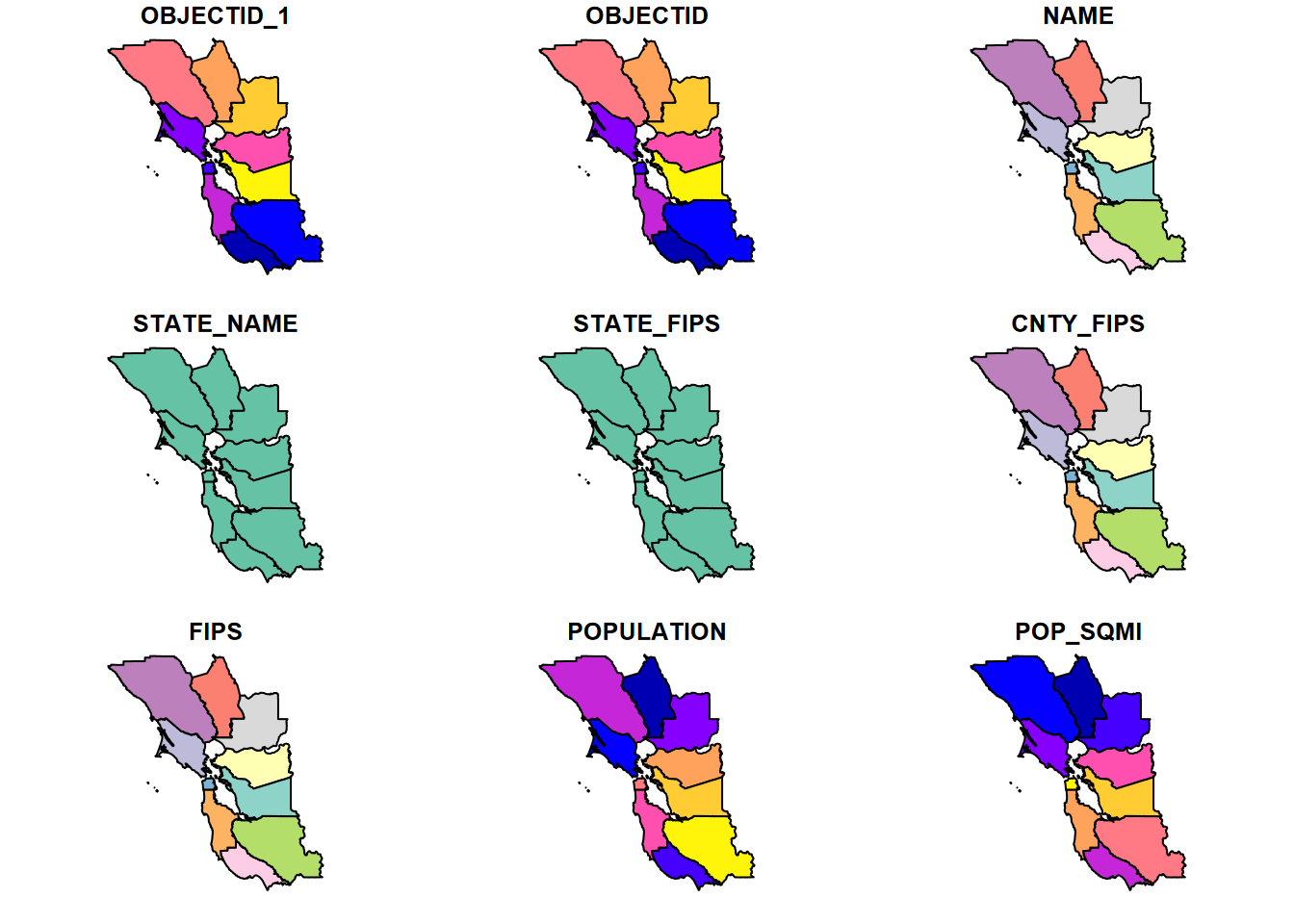FIGURE 5.8: `plot(BayAreaCounties)` by default shows all variables

But with just one variable, of course, it just produces a single map (Figure 5.9).

``plot(BayAreaCounties["POPULATION"])``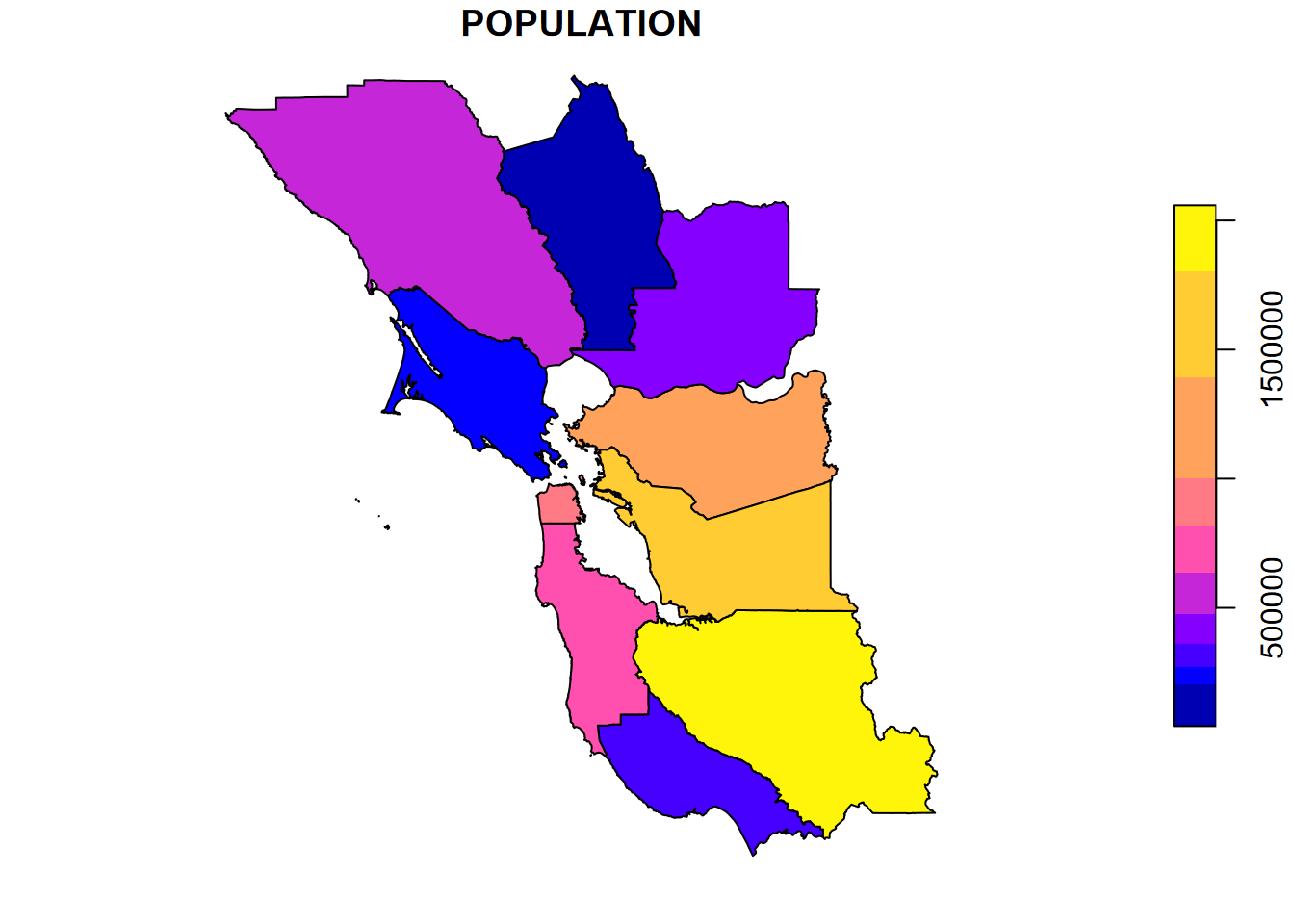FIGURE 5.9: plot(BayAreaCounties[“POPULATION”]

Notice that in the above map, we used the `[]` accessor. Why didn’t we use the simple `\$` accessor? Remember that plot() figures out what to do based on what you provide it. And there’s an important difference in what you get with the two accessors, which we can check with class():

``class(BayAreaCounties["POPULATION"])``
``##  "sf"         "data.frame"``
``class(BayAreaCounties\$POPULATION)``
``##  "numeric"``

You might see what you get with `plot(BayAreaCounties\$POPULATION)`, which is not very informative, since the object is just a numeric vector, while using `[]` accessor returns a spatial dataframe.

There’s a lot more we could do with the base R plot system, so we’ll learn some of these before exploring what we can do `ggplot2`, `tmap`, and `leaflet`. But first we need to learn more about building geospatial data.

We’ll use `st_as_sf()` for that, but we’ll need to specify the coordinate referencing system (CRS), in this case GCS.We’ll only briefly explore how to specify the CRS here. For a thorough coverage, please see Lovelace, Nowosad, and Muenchow (2019) .

### 5.1.5 Coordinate Referencing System

Before we try the next method for bringing in spatial data – converting data frames – we need to look at coordinate referencing systems (CRS). First, there are quite a few, with some spherical like the geographic coordinate system (GCS) of longitude and latitude, and others planar projections of GCS using mathematically defined projections such as Mercator, Albers Conformal Conic, Azimuthal, etc., and including widely used government-developed systems such as UTM (universal transverse mercator) or state plane. Even for GCS, there are many varieties since geodetic datums can be chosen, and for very fine resolution work where centimetres or even millimetres matter, this decision can be important (and tectonic plate movement can play havoc with tying it down.)

There are also multiple ways of expressing the CRS, either to read it or to set it. The full specification of a CRS can be displayed for data already in a CRS, with either `sf::st_crs` or `terra::crs`.

``st_crs(CA_counties)``
``````## Coordinate Reference System:
##   User input: WGS 84
##   wkt:
## GEOGCRS["WGS 84",
##     DATUM["World Geodetic System 1984",
##         ELLIPSOID["WGS 84",6378137,298.257223563,
##             LENGTHUNIT["metre",1]]],
##     PRIMEM["Greenwich",0,
##         ANGLEUNIT["degree",0.0174532925199433]],
##     CS[ellipsoidal,2],
##         AXIS["latitude",north,
##             ORDER,
##             ANGLEUNIT["degree",0.0174532925199433]],
##         AXIS["longitude",east,
##             ORDER,
##             ANGLEUNIT["degree",0.0174532925199433]],
##     ID["EPSG",4326]]``````

… though there are other ways to see the crs in shorter forms, or its individual properties :

``st_crs(CA_counties)\$proj4string``
``##  "+proj=longlat +datum=WGS84 +no_defs"``
``st_crs(CA_counties)\$units_gdal``
``##  "degree"``
``st_crs(CA_counties)\$epsg``
``##  4326``

There’s also `st_crs()\$Wkt` which you should try, but it creates a long continuous string that goes off the page, so doesn’t work for the book formatting.

``st_crs(CA_counties)\$Wkt # [Creates too long of a string for this book]``

So, to convert the sierra data into geospatial data with `st_as_sf`, we might either do it with the reasonably short PROJ format …

``````GCS <- "+proj=longlat +datum=WGS84 +no_defs +ellps=WGS84 +towgs84=0,0,0"
wsta = st_as_sf(sierraFeb, coords = c("LONGITUDE","LATITUDE"), crs=GCS)``````

… or with the even shorter EPSG code (which we can find by Googling), which can either be provided as text `"epsg:4326"` or often just the number, which we’ll use next.

### 5.1.6 Creating sf data from data frames

As we saw earlier, if your data frame has geospatial coordinates like `LONGITUDE` and `LATITUDE`

``names(sierraFeb)``
``````##  "STATION_NAME"  "COUNTY"        "ELEVATION"     "LATITUDE"
##  "LONGITUDE"     "PRECIPITATION" "TEMPERATURE"``````

… we have what we need to create geospatial data from it. Earlier we read in a series of vectors built in code with `c()` functions from 12 selected weather stations; this time we’ll use a data frame that has all of the Sierra Nevada weather stations (Figure 5.10).

``````wsta <- st_as_sf(sierraFeb, coords = c("LONGITUDE","LATITUDE"), crs=4326)
ggplot(data=wsta) + geom_sf(aes(col=ELEVATION))``````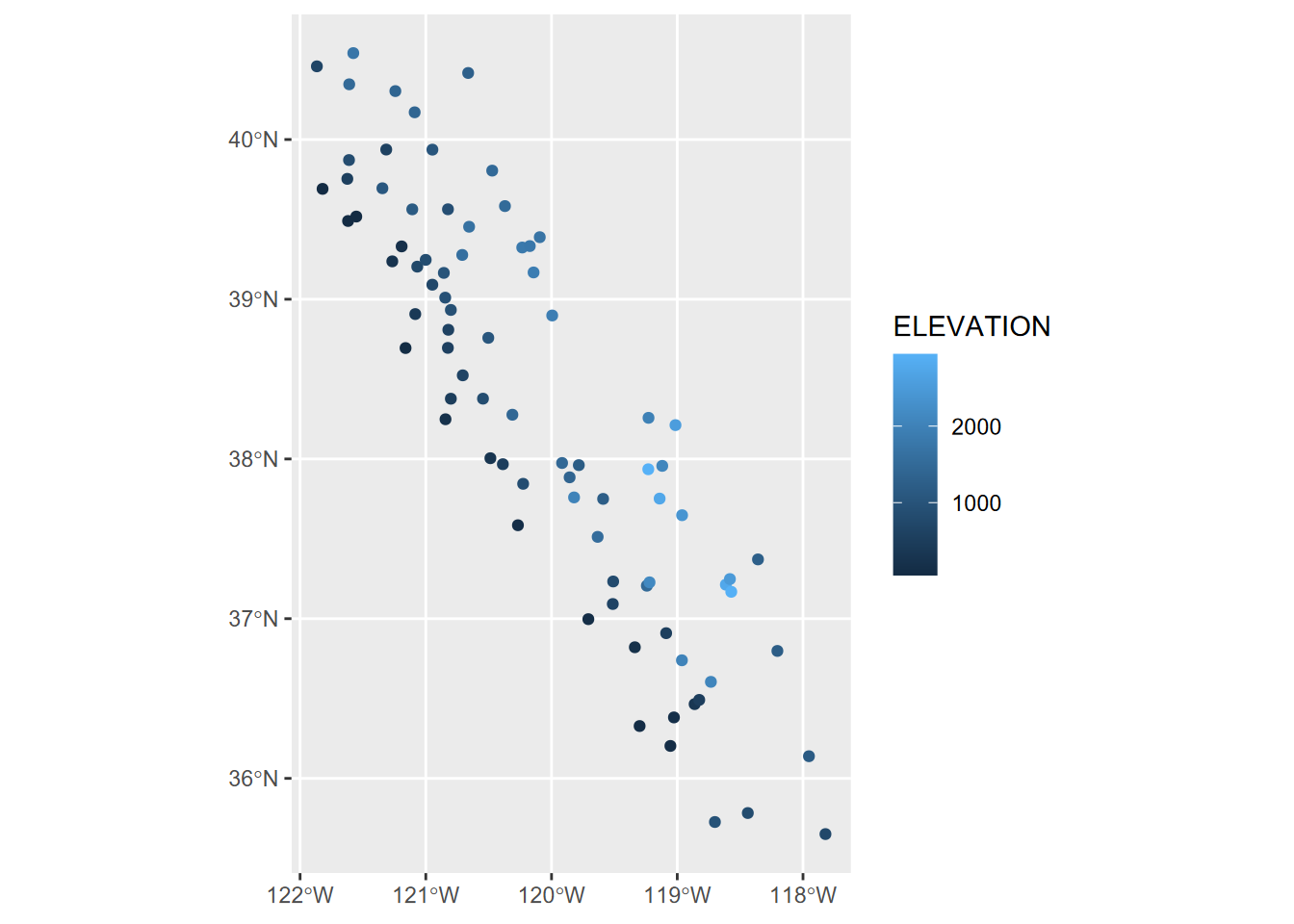FIGURE 5.10: Points created from data frame with coordinate variables

### 5.1.7Removing Geometry

There are many instances where you want to remove geometry from a sf data frame

• Some R functions run into problems with geometry and produce confusing error messages, like “non-numeric argument”

• You’re wanting to work with an sf data frame in a non-spatial way

One way to remove geometry :

`myNonSFdf <- mySFdf %>% st_set_geometry(NULL)`

## 5.2 Base R’s `plot()` with `terra`

As we’ve seen in the examples above, the venerable plot system in base R can often do a reasonable job of displaying a map. The terra package extends this a bit by providing tools for overlaying features on other features (or rasters using plotRGB), so it’s often easy to use (Figure 5.11).

``````library(terra)
plot(vect(wsta))
lines(vect(CA_counties), col="gray")``````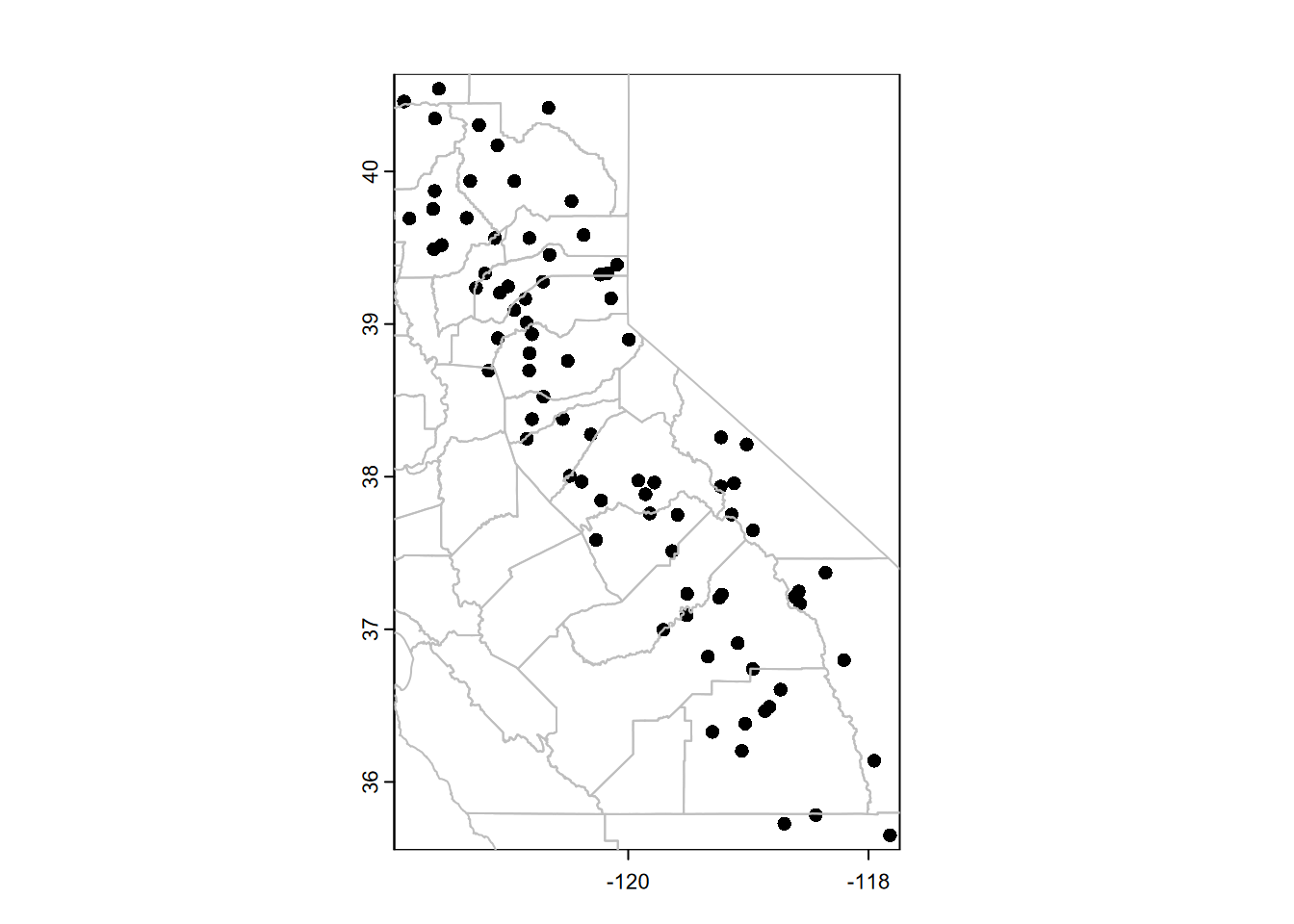FIGURE 5.11: Plotting SpatVector data with base R plot system

Note that we converted the sf data to `terra` `SpatVector` data with `vect()`, but the base R plot system can work with either `sf` data or `SpatVector` data from `terra`.

The `lines`, `points`, and `polys` functions (to name a few – see `?terra` and look at the Plotting section) will add to an existing plot. Alternatively, we could use plot’s `add=TRUE` parameter to add onto a plot that we’ve previously scaled with a plot. In this case, we’ll cheat and just use the first plot to set the scaling but then cover over that plot with other plots (Figure 5.12).

``````library(terra)
plot(vect(wsta)) # simple way of establishing the coordinates
# Then will be covered by the following: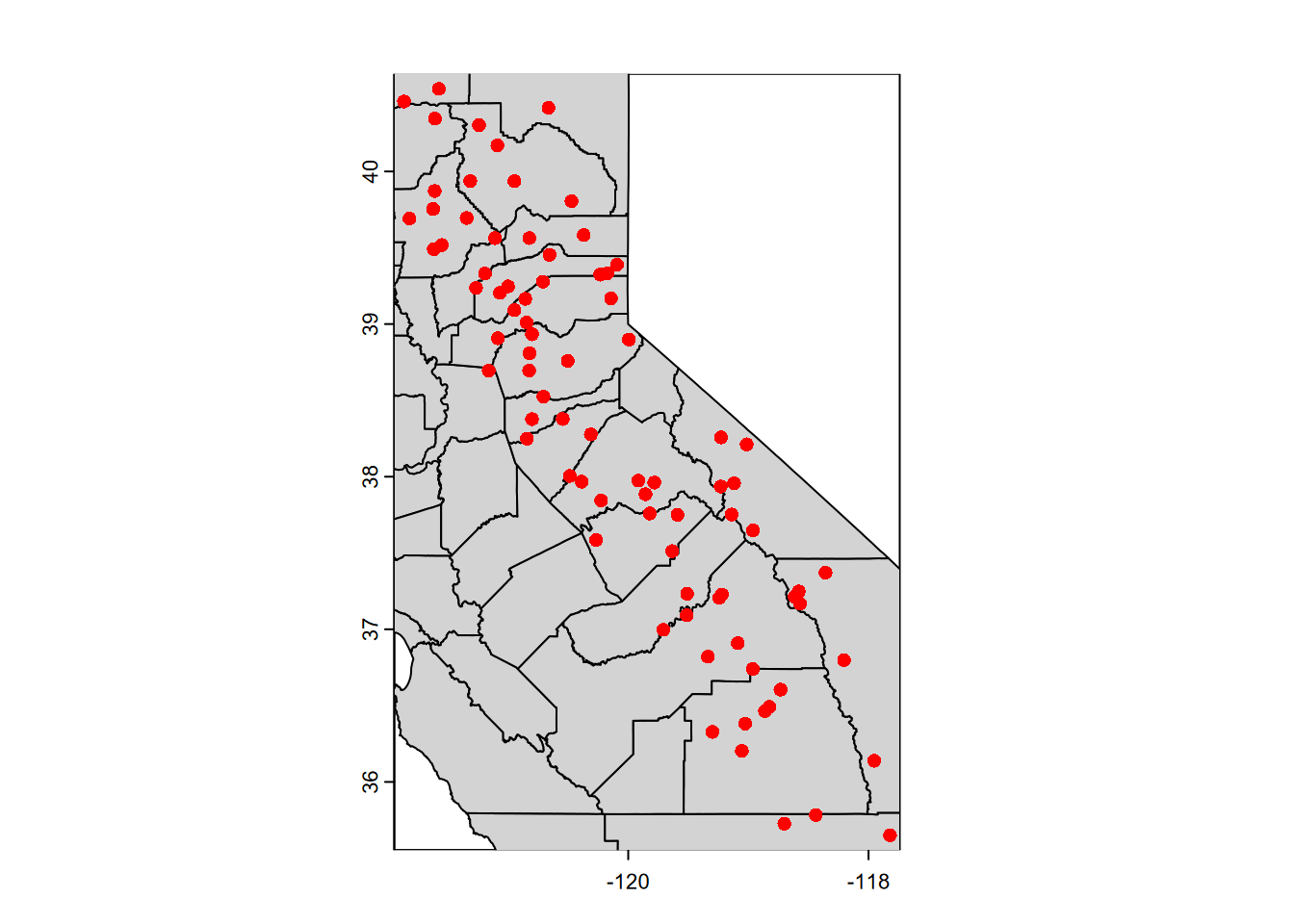FIGURE 5.12: Features added using `add=TRUE` in base R plot system

### 5.2.1 Using maptiles to create a basemap

For vector data, it’s often nice to display over a basemap by accessing raster tiles that are served on the internet by various providers. We’ll use the `maptiles` package to try displaying the `CA` and `NV` boundaries we created earlier. The `maptiles` package supports a variety of basemap providers, and I’ve gotten the following to work: `"OpenStreetMap"`, `"Stamen.Terrain"`, `"Stamen.TerrainBackground"`, `"Esri.WorldShadedRelief"`, `"Esri.NatGeoWorldMap"`, `"Esri.WorldGrayCanvas"`, `"CartoDB.Positron"`, `"CartoDB.Voyager"`, `"CartoDB.DarkMatter"`, `"OpenTopoMap"`, `"Wikimedia"`, however they don’t work at all scales and locations – you’ll often see an Error in grDevices, if so then try another provider – the default `"OpenStreetMap"` seems to work the most reliably (Figure 5.13).

``````library(terra); library(maptiles)
# Get the raster that covers the extent of CANV:
calnevaBase <- get_tiles(twostates, provider="OpenTopoMap")
st_crs(twostates)\$epsg``````
``##  4326``
``````plotRGB(calnevaBase)  # starts plot with a raster basemap
lines(vect(twostates), col="black", lwd=2)``````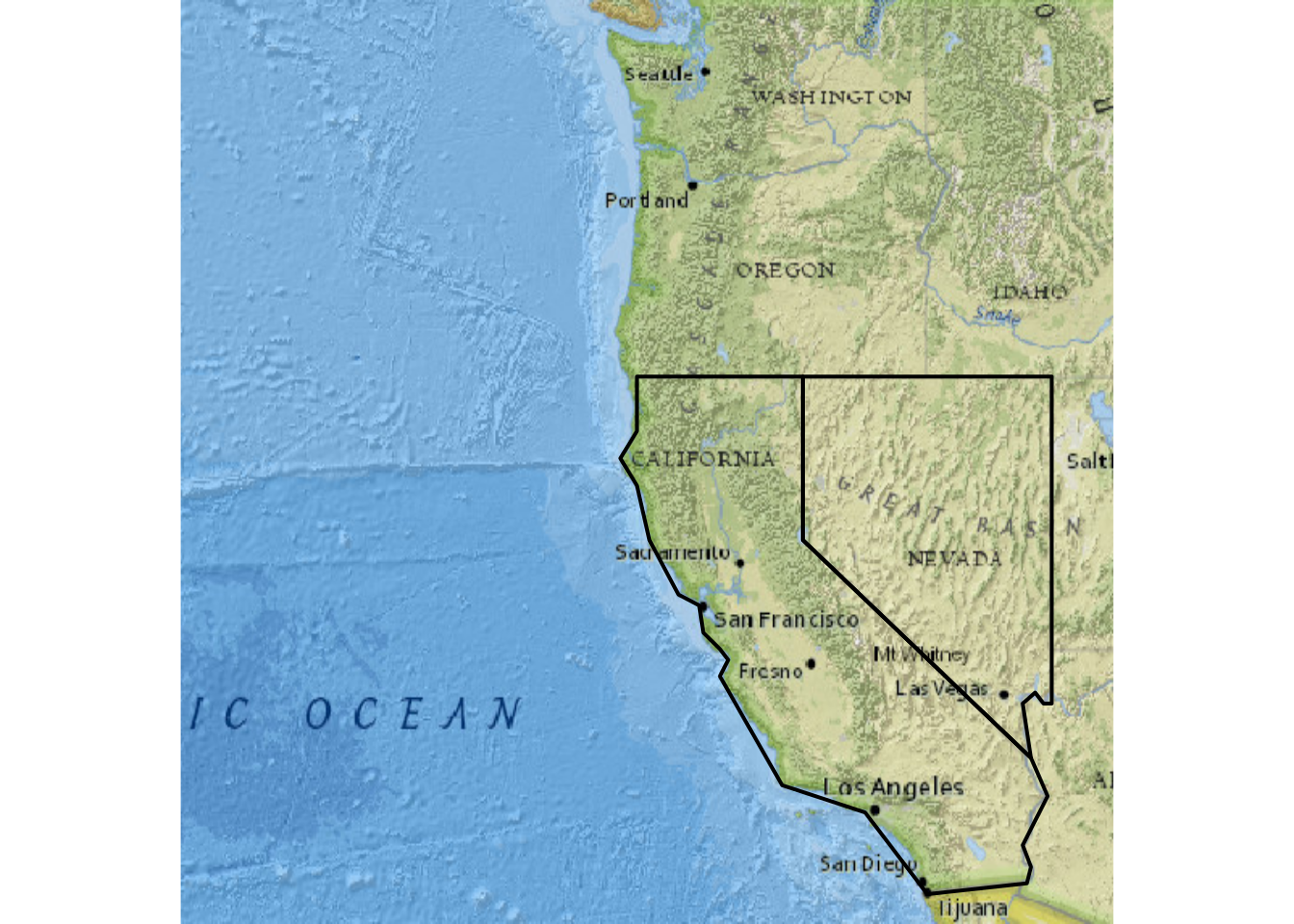FIGURE 5.13: Using maptiles for a base map

In the code above, we can also see the use of `terra` functions and parameters. Learn more about these by reviewing the code and considering:

terra functions: The `terra::vect()` function creates a `SpatVector` that works with `terra::lines` to display the boundary lines in `plot()`. (More on `terra` later, in the Raster section.)

parameters: Note the use of the parameter `lwd` (line width) from the plot system. This is one of many parameter settings described in `?par`. It defaults to 1, so 2 makes it twice as thick. You could also use lty (line type) to change it to a dashed line with `lty="dashed"` or `lty=2`.

And for the `sierraFeb` data, we’ll start with `st_as_sf` and the coordinate system (4326 for GCS), then use a `maptiles` basemap again, and the `terra::points` method to add the points (Figure 5.14).

``````library(terra); library(maptiles)
sierraFebpts <- st_as_sf(sierraFeb, coords = c("LONGITUDE", "LATITUDE"), crs=4326)
sierraBase <- get_tiles(sierraFebpts)
st_crs(sierraFebpts)\$epsg``````
``##  4326``
``````plotRGB(sierraBase)
points(vect(sierraFebpts))``````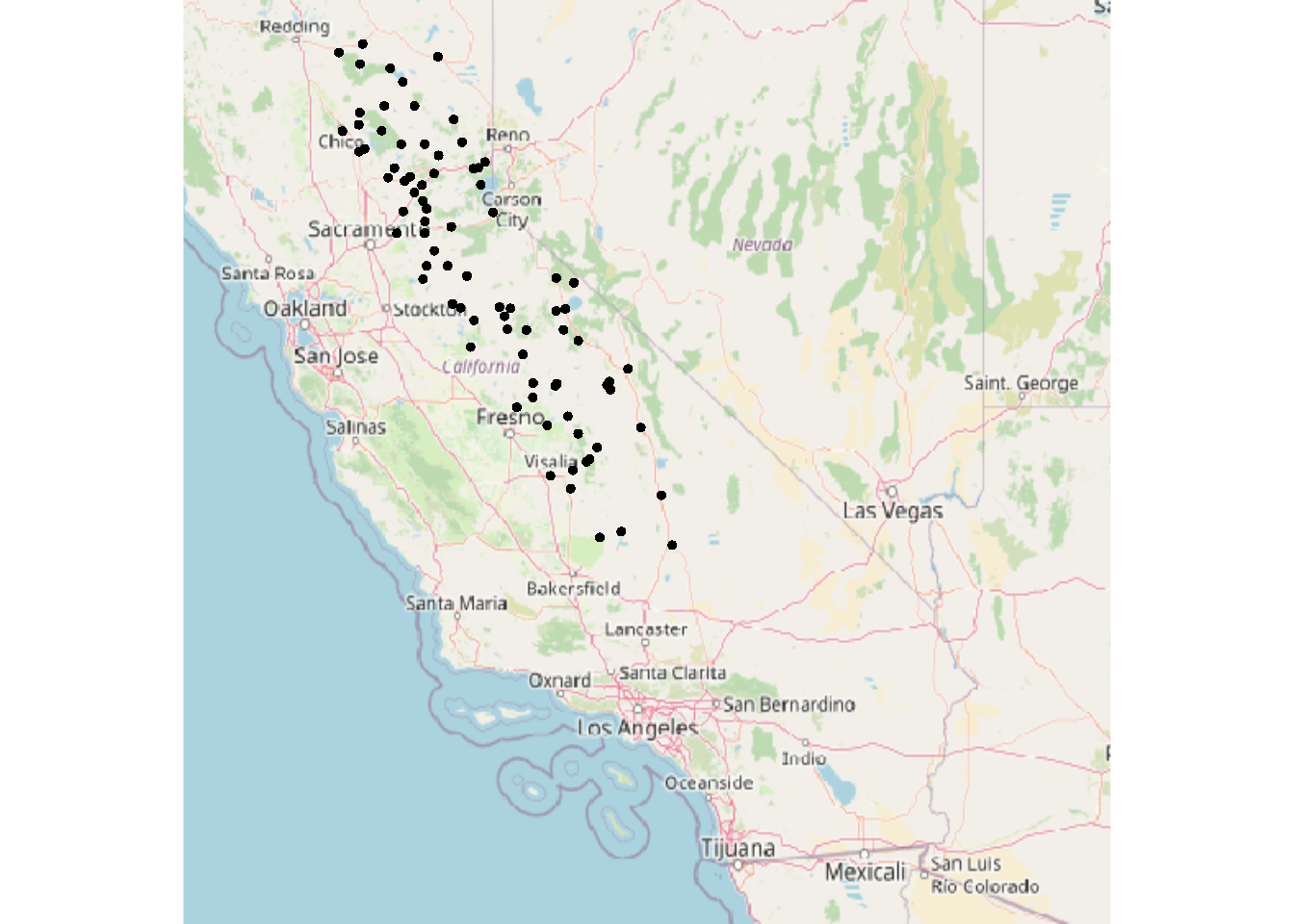FIGURE 5.14: Converted sf data for map with tiles

## 5.3 Raster data

Simple Features are feature-based, of course, so it’s not surprising that `sf` doesn’t have support for rasters. So we’ll want to either use the `raster` package or its imminent replacement, `terra` (which also has vector methods – see Hijmans (n.d.) and Lovelace, Nowosad, and Muenchow (2019)), and I’m increasingly using `terra` since it has some improvements that I find useful.

### 5.3.1 Building rasters

We can start by building one from scratch. The `terra` package has a `rast()` function for this, and creates an S4 (as opposed to the simpler S3) object type called a `SpatRaster`.

``````library(terra)
new_ras <- rast(ncol = 12, nrow = 6,
xmin = -180, xmax = 180, ymin = -90, ymax = 90,
vals = 1:72)``````

Formal S4 objects like `SpatRasters` have many associated properties and methods. To get a sense, type into the console `new_ras@ptr\$` and see what suggestions you get from the autocomplete system. To learn more about them, see Hijmans (n.d.) but we’ll look at a few of the key properties by simply entering the `SpatRaster` name in the console.

``new_ras``
``````## class       : SpatRaster
## dimensions  : 6, 12, 1  (nrow, ncol, nlyr)
## resolution  : 30, 30  (x, y)
## extent      : -180, 180, -90, 90  (xmin, xmax, ymin, ymax)
## coord. ref. : lon/lat WGS 84
## source      : memory
## name        : lyr.1
## min value   :     1
## max value   :    72``````
• The `nrow` and `ncol` dimensions should be familiar, and the `nlyr` tells us that this raster has a single layer (or band as they’re referred to with imagery), and it gives it the name `lyr.1`.
• The resolution is what you get when you divide 360 (degrees of longitude) by 12 and 180 (degrees of latitude) by 6, and the extent is what we entered to create it.
• But how does it know that these are degrees of longitude and latitude, as it appears to from the `coord. ref.` property? The author of this tool seems to let it assume GCS if it doesn’t exceed the limits of the planet: -180 to +180 longitude and -90 to +90 latitude.
• The source is in memory, since we didn’t read it from a file, but entered it directly. New rasters we might create from raster operations will also be in memory.
• The minimum and maximum values are what we used to create it.

The name `lyr.1` isn’t very useful, so let’s change the name with the `names` function, and then access the name with a `@ptr` property (you could also use `names(new_ras)` to access it, but I thought it might be useful to see the `@ptr` in action.)

``````names(new_ras) <- "world30deg"
new_ras``````
``````## class       : SpatRaster
## dimensions  : 6, 12, 1  (nrow, ncol, nlyr)
## resolution  : 30, 30  (x, y)
## extent      : -180, 180, -90, 90  (xmin, xmax, ymin, ymax)
## coord. ref. : lon/lat WGS 84
## source      : memory
## name        : world30deg
## min value   :          1
## max value   :         72``````

Simple feature data or `SpatVectors` can be plotted along with rasters using terra’s lines, polys, or points functions. Here we’ll use the `twostates` `sf` we created earlier (make sure to run that code again if you need it). Look closely at the map for our two states (Figure 5.15).

``````plot(new_ras, main=paste("CANV on",new_ras@ptr\$names))
CANV <- vect(twostates)
lines(CANV)``````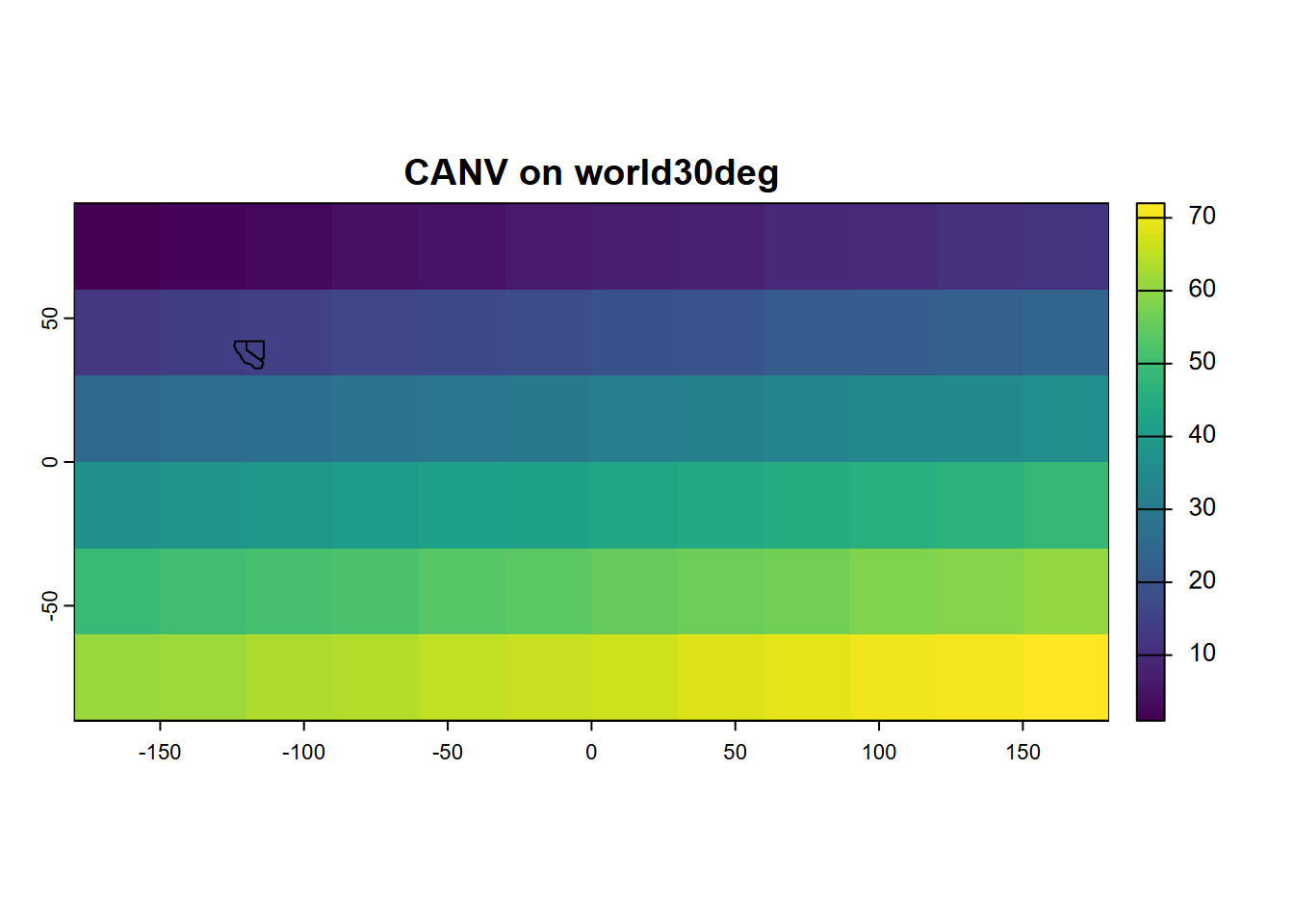FIGURE 5.15: Simple plot of a generated SpatRaster, with SpatVector added

Let’s plot some Shuttle Radar Topography Mission (SRTM) elevation data for the Virgin River Canyon at Zion National Park (Figure 5.16). First, the following required this method of installation:

``install.packages("spDataLarge", repos = "https://nowosad.github.io/drat/", type = "source")``
``````library(terra)
plot(rast(system.file("raster/srtm.tif", package="spDataLarge")))``````FIGURE 5.16: Shuttle Radar Topography Mission (SRTM) image of Virgin River Canyon area, southern Utah

## 5.4 ggplot2 for maps

The Grammar of Graphics is the gg of ggplot.

• Key concept is separating aesthetics from data
• Aesthetics can come from variables (using aes()setting) or be constant for the graph

• ggplot, as we have seen, and it continues to be enhanced (Figure 5.17)
• tmap (Thematic Maps) https://github.com/mtennekes/tmap Tennekes, M., 2018, tmap: Thematic Maps in R, Journal of Statistical Software 84(6), 1-39
``ggplot(CA_counties) + geom_sf()``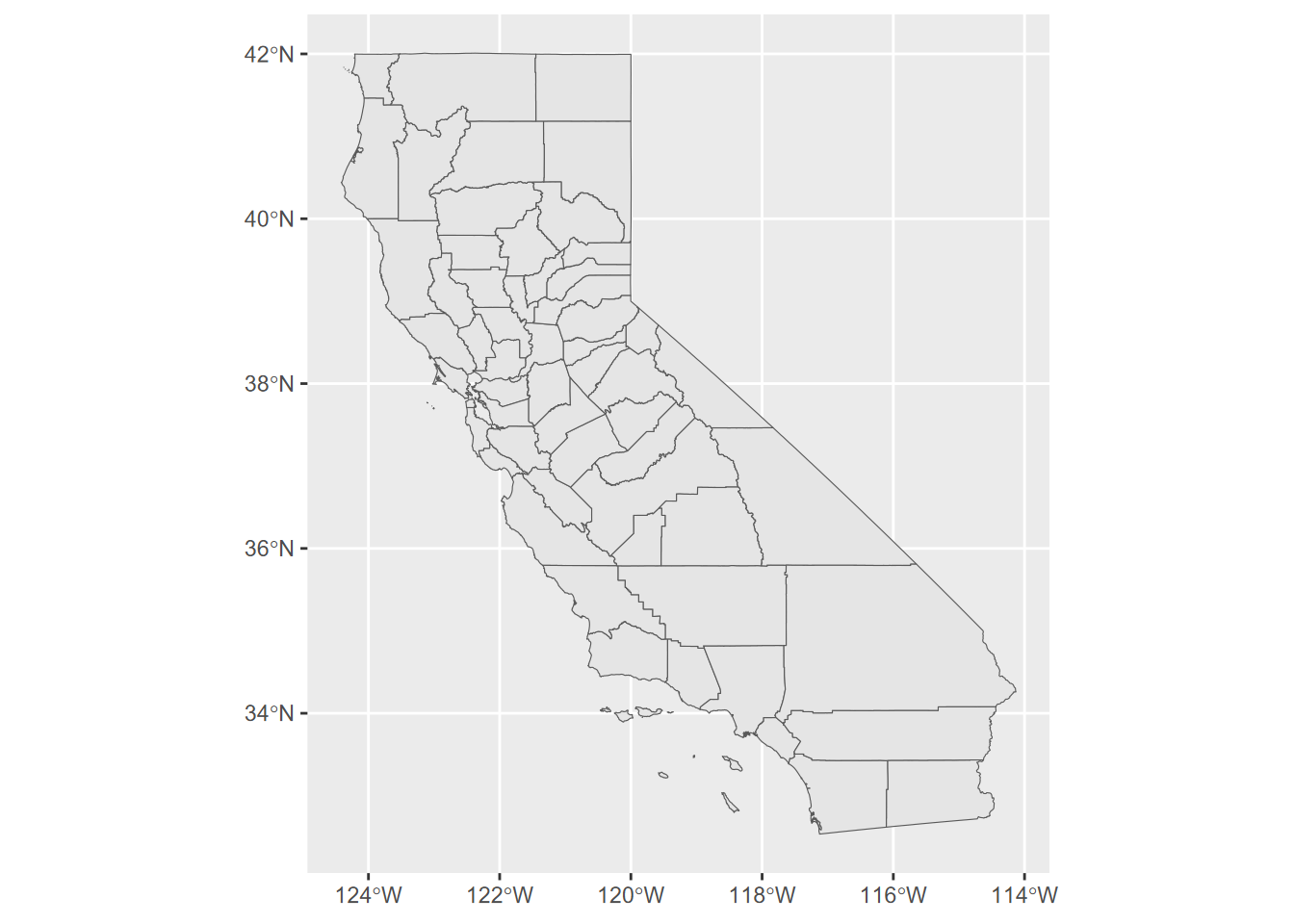FIGURE 5.17: simple ggplot map

Try `?geom_sf` and you’ll find that its first parameters is mapping with `aes()` by default. The data property is inherited from the ggplot call, but commonly you’ll want to specify data=something in your geom_sf call.

Another simple ggplot, with labels

Adding labels is also pretty easy using `aes()` (Figure 5.18).

``````ggplot(CA_counties) + geom_sf() +
geom_sf_text(aes(label = NAME), size = 1.5)``````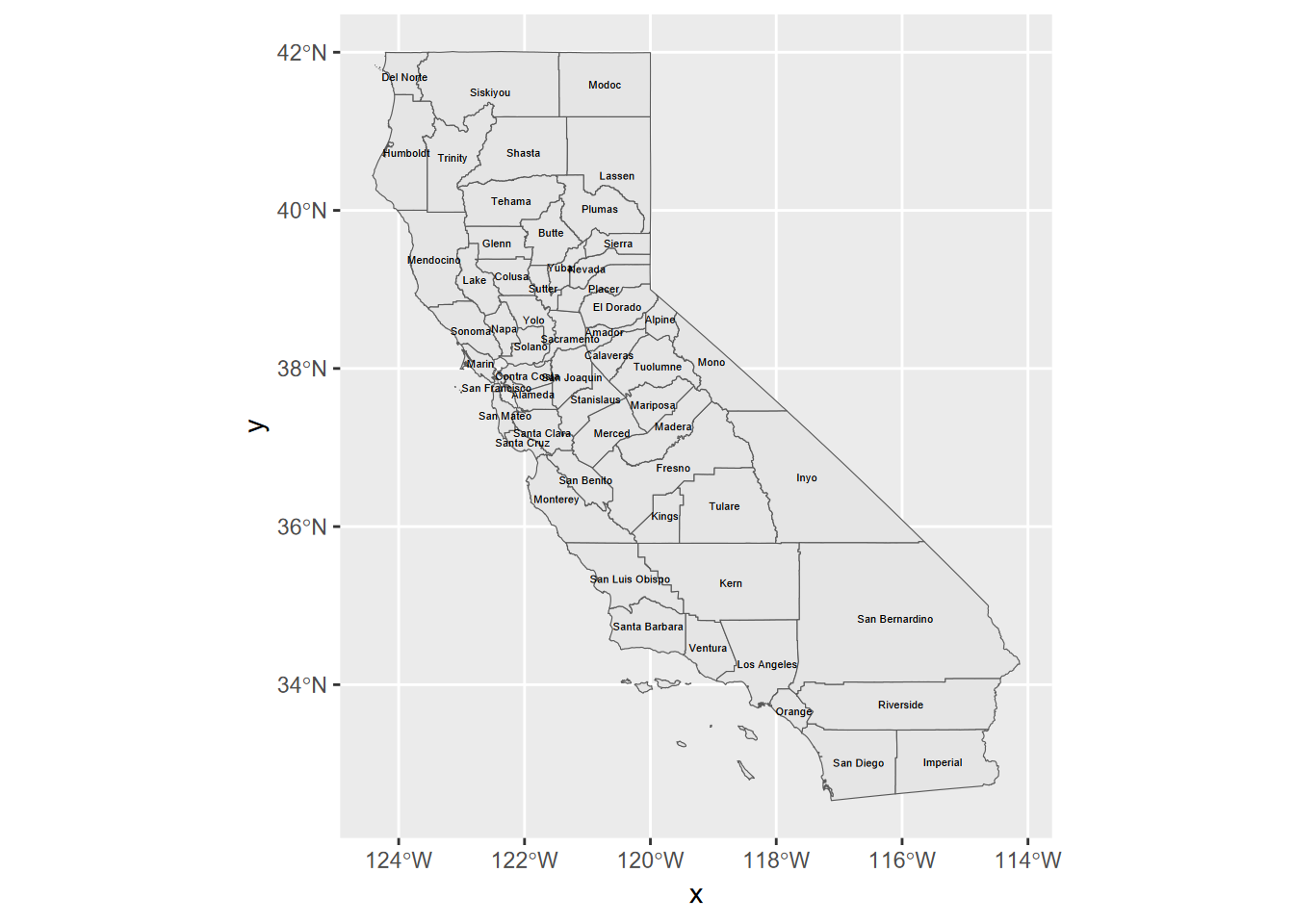And now with fill color, repositioned legend, and no “x” or “y” labels

The x and y labels are unnecessary since the graticule is provided, and for many maps there’s a better place to put the legend than what happens by default – for California’s shape, the legend goes best in Nevada (Figure 5.19).

``````ggplot(CA_counties) + geom_sf(aes(fill=MED_AGE)) +
geom_sf_text(aes(label = NAME), col="white", size=1.5) +
theme(legend.position = c(0.8, 0.8)) +
labs(x="",y="")``````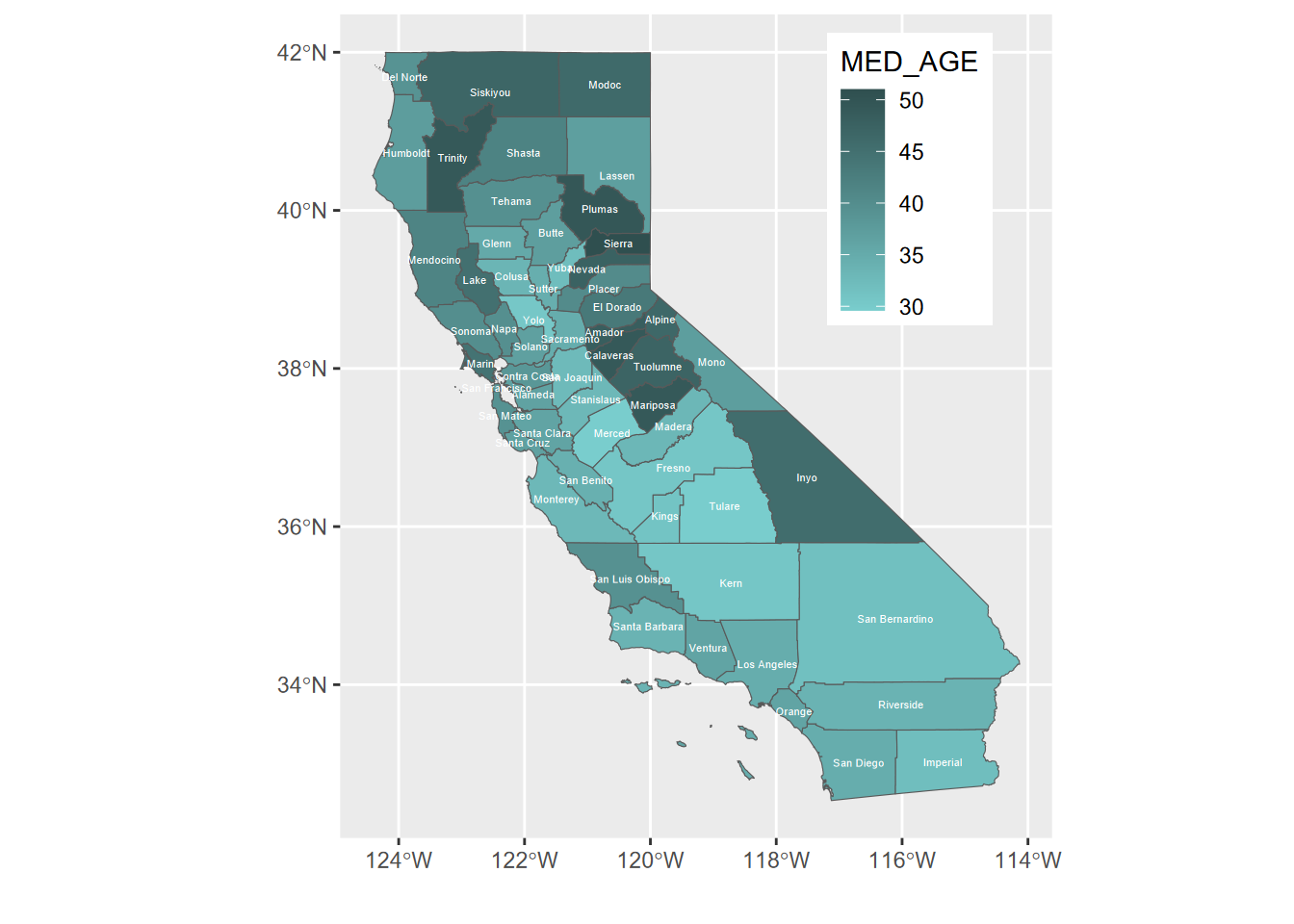FIGURE 5.19: repositioned legend

Map in ggplot2, zoomed into two counties

We can zoom into two counties by accessing the extent of an existing spatial dataset using `st_bbox()` (Figure 5.20).

``````library(tidyverse); library(sf); library(igisci)
census <- st_make_valid(BayAreaTracts) %>%
filter(CNTY_FIPS %in% c("013", "095"))
st_as_sf(coords = c("LONGITUDE", "LATITUDE"), crs=4326) %>%
st_join(census) %>%
filter(CNTY_FIPS %in% c("013", "095"),
(`5.1_FUGITIVE_AIR` + `5.2_STACK_AIR`) > 0)
bnd = st_bbox(census)
ggplot() +
geom_sf(data = BayAreaCounties, aes(fill = NAME)) +
geom_sf(data = census, color="grey40", fill = NA) +
geom_sf(data = TRI) +
coord_sf(xlim = c(bnd, bnd), ylim = c(bnd, bnd)) +
labs(title="Census Tracts and TRI air-release sites") +
theme(legend.position = "none")``````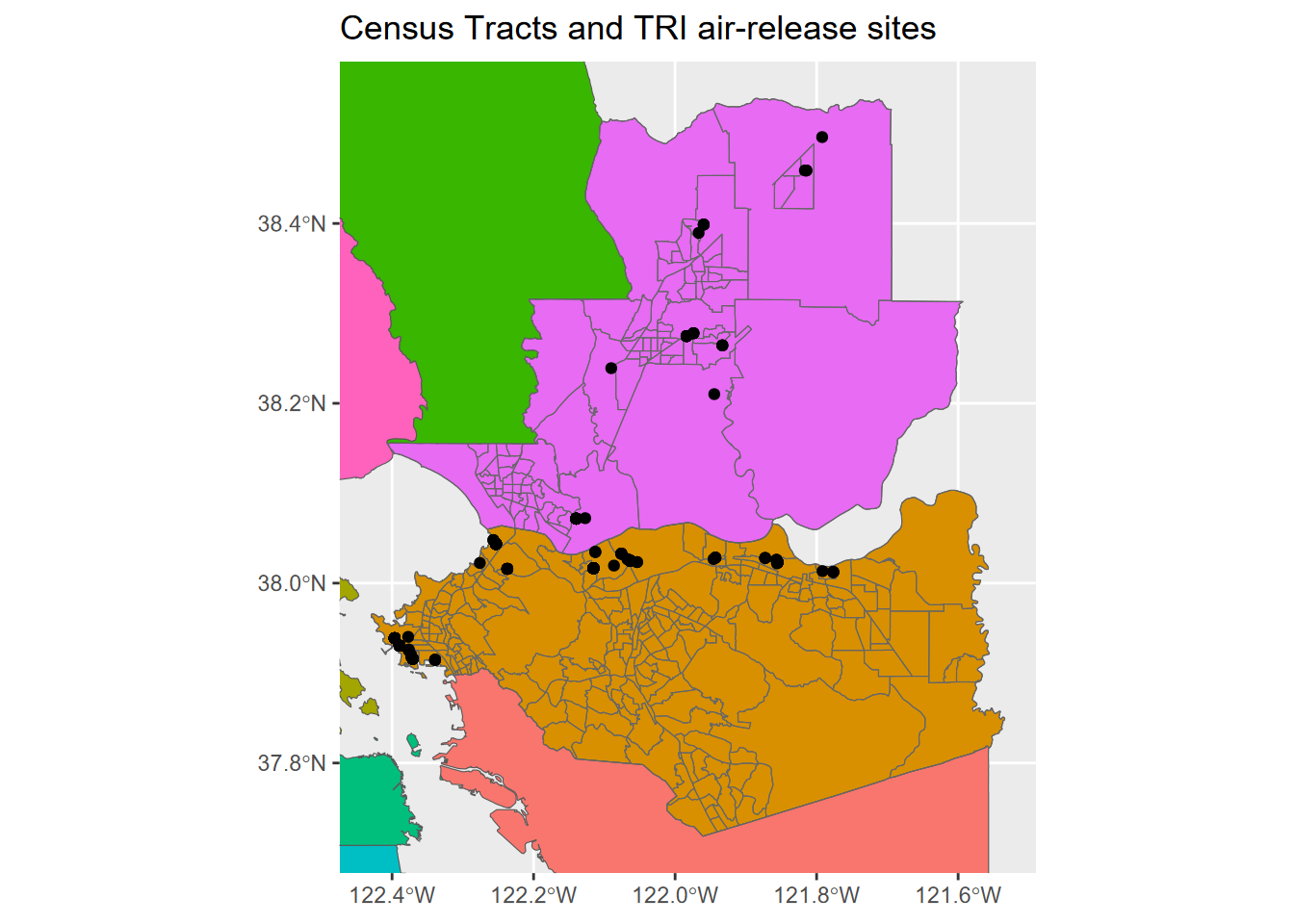FIGURE 5.20: Using bbox to zoom into 2 counties

### 5.4.1 Rasters in ggplot2

Raster display in `ggplot2` is currently a little awkward, as are rasters in general in the world of database-centric GIS where rasters don’t comfortably sit.

We can use a trick: converting rasters to a grid of points, to then be able to create Figure 5.21.

``````library(igisci)
library(tidyverse)
library(sf)
library(terra)
elevation <- rast(ex("marbles/elev.tif"))
elevpts <- st_as_sf(as.points(elevation))``````
``````ggplot() +
geom_sf(data = elevpts, aes(col=elev)) +
geom_sf(data = trails)``````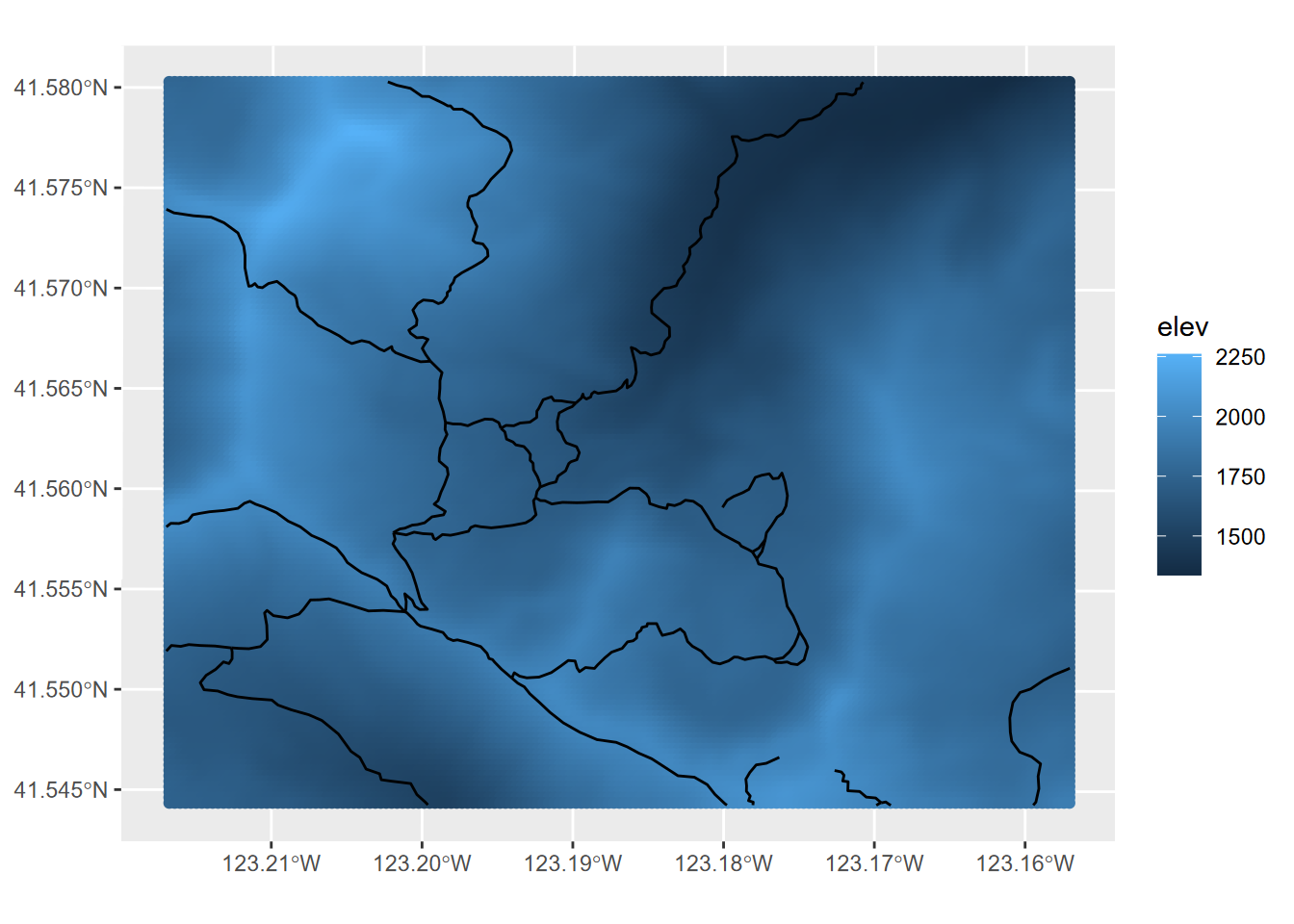FIGURE 5.21: Rasters displayed in ggplot by converting to points

Note that the raster name stored in the `elevation@ptr\$names` property is derived from the of the original source raster `elev.tif`, not from the object name we gave it, so we needed to specify `elev` for the variable to color from the resulting point features.

``names(elevation)``
``##  "elev"``

## 5.5 tmap

The tmap package provides some nice cartographic capabilities. We’ll use some of its capabilities, but for more thorough coverage, see Geocomputation with R Chapter 8 “Making Maps with R”: https://geocompr.robinlovelace.net/adv-map.html

The basic building block is `tm_shape(`data`)` followed by various layer elements such as tm_fill(). The tm_shape function can work with either features (Figure 5.22) or rasters.

``````library(spData)
library(tmap)
m <- tm_shape(world) + tm_fill() + tm_borders()
m``````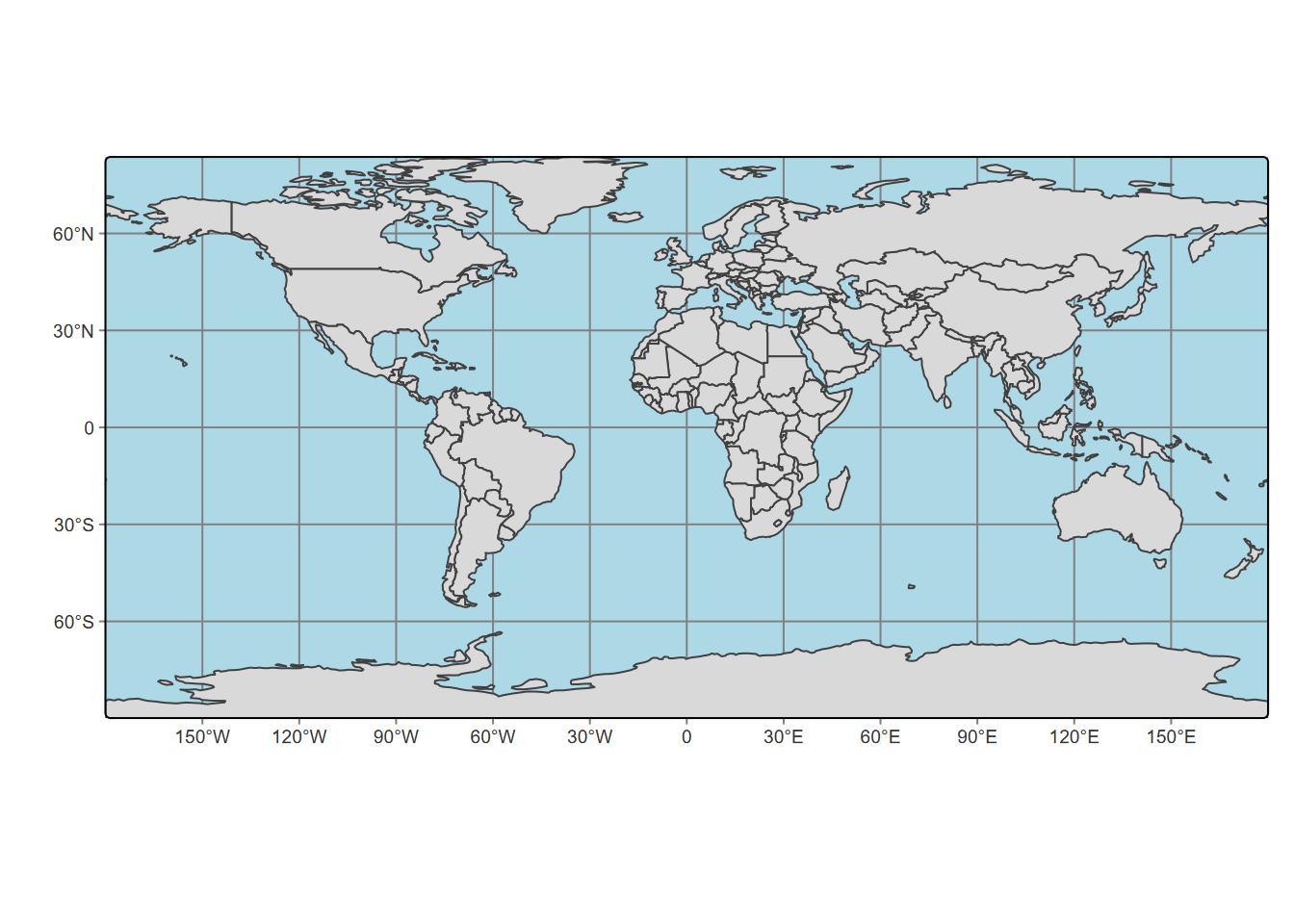FIGURE 5.22: tmap of the world

Color by variable

As with plot and ggplot2, we can reference a variable to provide a range of colors for features we’re plotting, such as coloring polygon fills to create a choropleth map (Figure 5.23).

``````library(sf)
library(tmap)
tm_shape(st_make_valid(BayAreaTracts)) + tm_fill(col = "MED_AGE")``````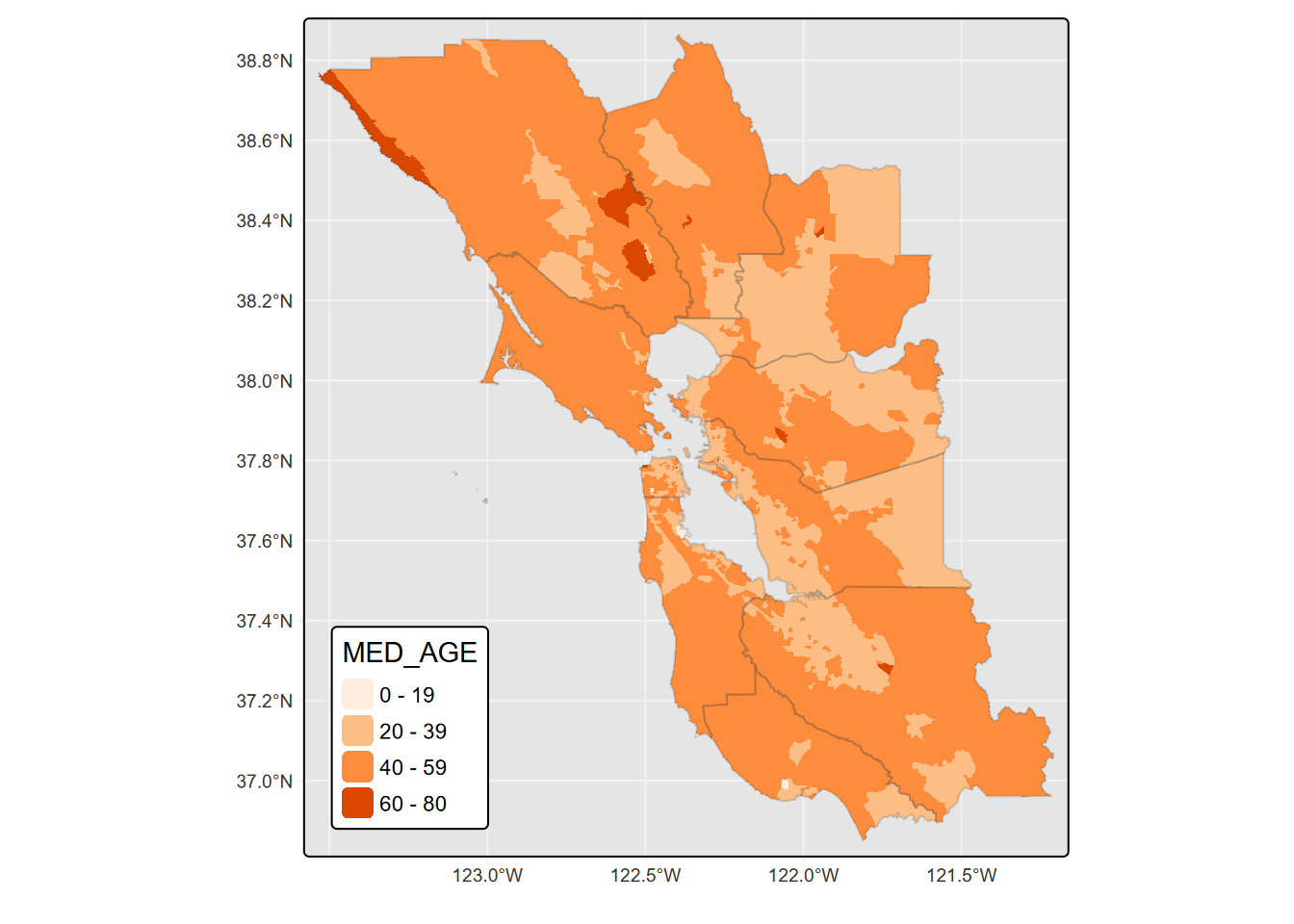FIGURE 5.23: tmap fill colored by variable

tmap of sierraFeb with hillshade and point symbols

We’ll use a raster hillshade as a basemap using `tm_raster`, zoom into an area with a bounding box (from `st_bbox`), include county boundaries with `tm_borders`, and color station points with temperatures with `tm_symbols` (Figure 5.24).

``````library(terra) # alt: library(raster)
tmap_mode("plot")
tmap_options(max.categories = 8)
sierra <- st_as_sf(sierraFeb, coords = c("LONGITUDE", "LATITUDE"), crs = 4326)
hillsh <- rast(ex("CA/ca_hillsh_WGS84.tif"))   # alt: hillsh <- raster(...)
bounds <- st_bbox(sierra)
tm_shape(hillsh,bbox=bounds)+
tm_raster(palette="-Greys",legend.show=FALSE,n=10) +
tm_shape(sierra) +
tm_symbols(col="TEMPERATURE", palette=c("blue","red"), style="cont",n=8) +
tm_shape(st_make_valid(CA_counties)) + tm_borders() +
tm_legend() +
tm_layout(legend.position=c("RIGHT","TOP"))``````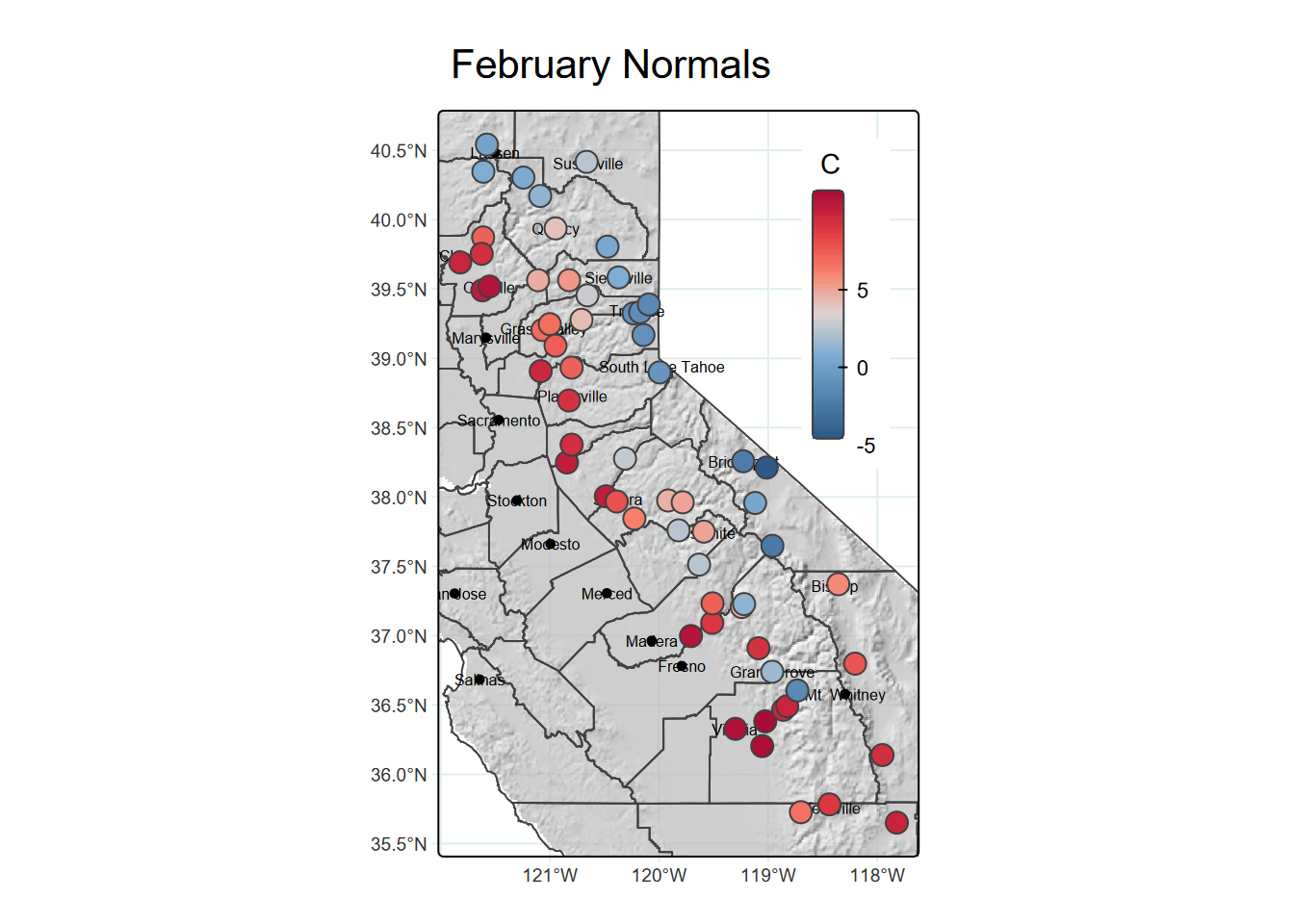FIGURE 5.24: hillshade, borders and point symbols in tmap

Color (rgb) basemap

Earlier we looked at creating a basemap using `maptiles`. These are also supported in tmap using the `tm_rgb` (Figure 5.25).

``````library(tmap); library(maptiles)
# The following gets the raster that covers the extent of CA & NV [twostates]
calnevaBase <- get_tiles(twostates, provider="OpenTopoMap")# g
tm_shape(calnevaBase) + tm_rgb() +
tm_shape(twostates) + tm_borders(lwd=3)``````FIGURE 5.25: Two western states with a basemap in tmap

## 5.6 Interactive Maps

The word “static” in “static maps” isn’t something you would have heard in a cartography class 30 years ago, since essentially all maps then were static. Very important in designing maps was, and still is, considering your audience and their perception of symbology.

• Figure-to-ground relationships assume “ground” is a white piece of paper (or possibly a standard white background in a pdf), so good cartographic color schemes tend to range from light for low values to dark for high values.
• Scale is fixed and there are no “tools” for changing scale, so a lot of attention must be paid to providing scale information.
• Similarly, without the ability to see the map at different scales, inset maps are often needed to provide context.

Interactive maps change the game in having tools for changing scale, and always being “printed” on a computer or device where the color of the background isn’t necessarily white. We are increasingly used to using interactive maps on our phones or other devices, and often get frustrated not being able to zoom into a static map. However, as we’ll see there are trade-offs in that interactive maps don’t provide the same level of control on symbology for what we want to put on the map, but instead depend a lot on basemaps for much of the cartographic design, generally limiting the symbology of data being mapped on top of it.

### 5.6.1 Leaflet

We’ll come back to tmap to look at its interactive option, but we should start with a very brief look at the package that it uses when you choose interactive mode: leaflet. The R leaflet library itself translates to Javascript and its Leaflet library, which was designed to support “mobile-friendly interactive maps” (https://leafletjs.com). We’ll also look at another R package that translates to leaflet: mapview.

Interactive maps tend to focus on using basemaps for most of the cartographic design work, and quite a few are available. The only code required to display the basemap is `addTiles()` which will display the default `OpenStreetMap` in the area where you provide any features, typically with `addMarkers()`. This default basemap is pretty good to use for general purposes, especially in urban areas where OpenStreetMap contributors (including a lot of former students I think) have provided a lot of data.

You can specify additional choices using `addProviderTiles()`, and use a layers control with the choices provided as `baseGroups`. You have access to all of the basemap provider names with the vector `providers`, and to see what they look like in your area, explore http://leaflet-extras.github.io/leaflet-providers/preview/index.html where you can zoom into an area of interest and select the base map to see how it would look in a given zoom (Figure ??).

``````library(leaflet)
leaflet() %>%
popup="Institute for Geographic Information Science, SFSU") %>%
baseGroups = c("OpenStreetMap","OpenTopoMap",
"Esri.WorldImagery","Esri.NatGeoWorldMap"))``````

With an interactive map, we do have the advantage of a good choice of base maps and the ability to resize and explore the map, but symbology is more limited, mostly just color and size, with only one variable in a legend. Note that interactive maps display in the Viewer window of RStudio or in R Markdown code output as you see in the html version of this book.

For a lot more information on the leaflet package in R, see:

### 5.6.2 Mapview

In the mapview package, either `mapview` or `mapView` produces perhaps the simplest interactive map display where the view provides the option of changing basemaps, and just a couple of options make it a pretty useful system for visualizing point data, so for environmental work involving samples, is a pretty useful exploratory tool.

Try the following code. Mapview doesn’t create figure headings (though leaflet does), so we won’t include the output here, but as you can see the mapview code is very simple.

``````library(sf); library(mapview)
sierra <- st_as_sf(sierraFeb, coords = c("LONGITUDE", "LATITUDE"), crs = 4326)
mapview(sierra, zcol="TEMPERATURE", popup = TRUE)``````

### 5.6.3 tmap (view mode)

You can change to an interactive mode with tmap by using `tmap_mode("view")` so you might think that you can do all the great things that `tmap` does in normal plot mode here, but as we’ve seen, interactive maps don’t provide the same level of symbology. The view mode of tmap, like mapview, is just a wrapper around leaflet, so we’ll focus on the latter. The key parameter needed is `tmap_mode` which must be set to `"view"` to create an interactive map.

``````library(tmap); library(sf)
tmap_mode("view")
tmap_options(max.categories = 8)
sierra <- st_as_sf(sierraFeb, coords = c("LONGITUDE", "LATITUDE"), crs = 4326)
bounds <- st_bbox(sierra)
#sierraMap <-
tm_basemap(leaflet::providers\$Esri.NatGeoWorldMap) +
tm_shape(sierra) + tm_symbols(col="TEMPERATURE",
palette=c("blue","red"), style="cont",n=8,size=0.2) +
tm_legend() +
tm_layout(legend.position=c("RIGHT","TOP"))``````
``````#sierraMap
#tmap_leaflet(sierraMap)``````

One nice feature of tmap view mode (and mapview) is the ability to select the basemap interactively using the layers symbol. There are lots of basemap available with leaflet (and thus with tmap view). To explore them, see http://leaflet-extras.github.io/leaflet-providers/preview/index.html but recognize that not all of these will work everywhere; many are highly localized or may only work at certain scales.

The following map using tmap could presumably be coded in leaflet, but tmap makes it a lot easier. We’ll use it to demonstrate picking the basemap, which really can help in communicating our data with context (Figure ??).

``````library(sf); library(tmap); library(igisci)
tmap_mode = "view"
tmap_options(basemaps=c(Topo="OpenTopoMap", Imagery = "Esri.WorldImagery",
NatGeo="Esri.NatGeoWorldMap"))
``knitr::include_graphics(here::here("img","tMapBaseMaps.png"))``
``````mblCO2map <- tm_basemap() +
tm_shape(geology) + tm_fill(col="CLASS", alpha=0.5) +
tm_shape(soilCO2) + tm_symbols(col="CO2_",
palette="viridis", style="cont",n=8,size=0.6) +
tm_legend() +
tm_layout(legend.position=c("RIGHT","TOP"))
mblCO2map``````
``#tmap_leaflet(mblCO2map)``

### 5.6.4 Interactive mapping of individual penguins abstracted from a big dataset

In a study of spatial foraging patterns by Adélie penguins (Pygoscelis adeliae), Ballard et al. (Ballard et al. (2019)) collected data over five austral summer seasons (parts of December and January in 2005-06, 2006-07, 2007-08, 2008-09, 2012-13) period using SPLASH tags that combine Argos satellite tracking with a time-depth recorder, with 11,192 observations. The 24 SPLASH tags were re-used over the period of the project with a total of 162 individual breeding penguins, each active over a single season.

Our code takes advantage of the abstraction capabilities of base R and `dplyr` to select an individual penguin from the large data set and prepare its variables for useful display. Then the interactive mode of tmap works well for visualizing the penguin data – both the cloud of all all observations and the focused individual – since we can zoom in to see the locations in context, and basemaps can be chosen. The `tmap` code colors the individual penguin’s locations based on a decimal day derived from day and time. While interactive view is more limited in options, it does at least support a legend and title (Figure ??).

``````library(sf); library(tmap); library(tidyverse); library(igisci)
obs <- st_as_sf(filter(sat_table,include==1), coords=c("lon","lat"), crs=4326)
uniq_ids <- unique(obs\$uniq_id) # uniq_id identifies an individual penguin
onebird <- obs %>%
filter(uniq_id==uniq_ids[sample.int(length(uniq_ids), size=1)]) %>%
mutate(decimalDay = as.numeric(day) + as.numeric(hour)/24)``````
``knitr::include_graphics(here::here("img","mapsPenguins.png"))``
``````tmap_mode = "view"
tmap_options(basemaps=c(Terrain = "Esri.WorldTerrain",
Imagery = "Esri.WorldImagery",
OceanBasemap = "Esri.OceanBasemap",
Topo="OpenTopoMap",
Ortho="GeoportailFrance.orthos"))
penguinMap <- tm_basemap() +
tm_shape(obs) + tm_symbols(col="gray", size=0.01, alpha=0.5) +
tm_shape(onebird) + tm_symbols(col="decimalDay",
palette="viridis", style="cont",n=8,size=0.6) +
tm_legend() +
tm_layout()
tmap_leaflet(penguinMap)``````